# Rasnik Analysis

© 2007-2020, Kevan Hashemi, Brandeis University

## Contents

Introduction
Source Code
Execution Time
Derivative Images
Fourier Transform
Smoothed Peaks
Line Fitting
Code Squares
Display
Blank Images
Random Boundaries
Pre-Filtering
Measurement Precision
Simulated Images
Analysis Failure
Skewed Images
Large Rotations
Subroutines
Data Types
Calling from C

## Introduction

Note: Our descriptin of the C interface with the LWDAQ analysis library applies to LWDAQ 9 or earlier. We have yet to prepare and write up the C interface of our LWDAQ 10 routines.

This report describes our work on our rasnik (Red Alignment System of NIKhef) image analysis program. In detail, the report applies to the rasnik analysis provided by LWDAQ 8.5.9+. We describe how the analysis works, how to analyze rasnik image with our LWDAQ Software, and how to link your own programs to our analysis libraries so you can build rasnik analysis into your own applications. Below is a rasnik image. The pattern is a chessboard with some squares flipped from black to white, and others flipped from white to black.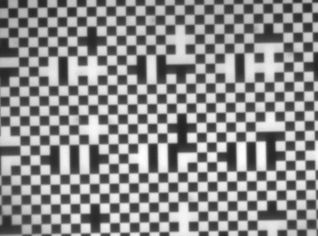Figure: A Rasnik Image.

A rasnik instrument consists of a mask, lens, and image sensor. The lens focuses an image of the mask onto the image sensor. The figure above is an example of such an image. The mask is a chessboard-like pattern on a piece of glass, illuminated from behind by diffuse light. The image sensor might be a CCD, such as the TC255P we use in the ATLAS end-cap alignment system, or the CMOS sensors used by NIKHEF in the ATLAS barrel alignment system, or the TC237B we will use in ATLAS upgrades.

When the three components of a rasnik instrument move with respect to one another, the mask image moves or changes size on the image sensor. The mask is larger than the image sensor. Typical masks are 30 mm square. Our image sensors are only 3.4 mm × 2.4 mm. When the three components are lined up perfectly, and the lens is placed so as to give a sharp image like the one we show above, the center of the mask will be focused onto the center of the image sensor. The mask can move almost half its width in any direction, and still cover the image sensor entirely with the mask pattern. The unusual squares in a rasnik pattern contain codes that allow us to determine which part of the mask is focused on the image. We call these squares code squares. The purpose of rasnik image analysis is to determine the point in the mask that is focused onto the center of the image sensor (or any other reference point in the image sensor you care to choose, but we and NIKHEF prefer using the center). We call this point in the mask the rasnik position.

The smallest mask squares we use are 85 μm wide. The largest are 340 μm wide. When the lense is not equidistant from both mask and image sensor, the mask image can be magnified or de-magnified. We choose a mask square size that gives between twenty and thirty squares across the image sensor, so that there are plenty of edges for our anlysis to find, and plenty of code squares.

With a sharp image and less than a meter between the mask and image sensor, we can determine the rasnik position to a fraction of a micron. With sixteen meters between the mask and image sensor, the image becomes blurred by diffraction at the lens, and moves around because of turbulence in air. We find that our 16-m precision is more like ten microns. If you place a rasnik in an evacuated tube, as NIKHEF has done in a test stand at CERN recently, the rasnik resolution over a hundred meters is less than a micron.

For an explanation of the rasnik from its creators, see the Rasnik Home Page. For our work with rasnik instruments, see the following documents.

The Optical Alignment System of the
ATLAS Muon Spectrometer Endcaps
Description of ATLAS end-cap alignment system,
including description of our rasnik instruments.
RASNIK Image Analysis with
a Steepest Ascent Algorithm
Our original, now obsolete, rasnik analysis (1997).
Pixel CCD RASNIK The image sensors we use in our rasnik instruments (1997).
Pixel CCD RASNIK DAQ Our original, now obsolete, data acquisition system (1997).
RASNIK Depth of Field How to balance focus and diffraction to maximize depth of field (1997).
LWDAQ User Manual Our current data acquisition system (2004 to present).
Rasnik Instrument Software Our LWDAQ rasnik instrument graphical user interface (2004 to present).
Rasnik Analysis Software Our Pascal rasnik image analysis code (2004 to present).
Table: Existing Brandeis University HEP Rasnik Documents.

Our analysis finds the rasnik pattern and determines the rasnik position. It marks the pattern it finds on the images, as you can see below, in which we show the results of analysis for the same image shown above.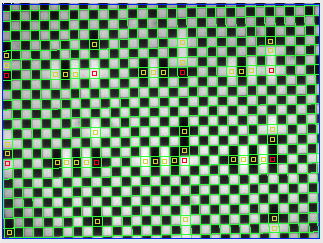Figure: Rasnik Image with Analysis Results Overlayed.

We invite you to download our LWDAQ Software here. The software runs on MacOS, Linux, and Windows. You will find installation instructions for each platform here. Install and run the software and open the Rasnik Instrument in the Instrument menu. Press Acquire. The software will try to capture a rasnik image from our demonstration stand, analyze it, and display the results. Maybe the analysis fails, in which case increase the daq_flash_seconds to make the image brighter, and try again. Or you can change image_source to "file", and press Acquire. You will see all our sample images appearing, and their analysis results being printed in the Rasnik Instrument text window. Press the Info button and set analysis_show_fitting to "1000". Press Acquire again. You will see the analysis pausing for one second at a time, and at each pause, you will see a different stage of the rasnik analysis. Set analysis_show_fitting to "-1" and the analysis will pause at each stage until you press a button.

By default, the Rasnik Instrument produces a single line of numbers like this:

`Rasnik_benchmark.gif 32535.26 24236.77 0.469865 0.470965 8.519 0.554 120.0 10.0 4 1720.0 1220.0 0.464 0.019 1.140`

The more verbose result from the same instrument looks like the print-out below, and includes a description of each parameter. The exact numbers are not the same as above because we acquired a second image and analyzed it to obtain the output.

```Rasnik_benchmark.gif
Image Magnification X (mm/mm): 0.469865
Image Magnification Y (mm/mm): 0.470965
Measurement Precision (um in mask): 0.554
Pixel Size (um): 10.0
Orientation Code (the code chosen by analysis): 4
Reference Point X (um from left edge of CCD): 1720.0
Reference Point Y (um from top edge of CCD): 1220.0

For an explanation of the reference and orientation codes, see our description of the Rasnik Instrument. For now, we will say that the reference code determines the reference point on the image sensor. When we compare rasnik analysis programs, our tradition is to use the top-left corner of the top-left pixel as the reference point, because this point is easy to define when we have images of varying sizes. It's the top-left corner of pixel (0,0).

A rasnik measurement is the determination of the point in the mask that is projected by the optics onto the reference point in the image sensor. It may be that the reference point is not a light-sensitive pixel. It may be part of a black left-hand border in the image. But we can still calculate which point in the mask would have been projected onto the reference point if the reference point were a light-sensitive pixel. The rasnik measurement consists of the x and y coordinates of a point in the mask, the magnification of the mask image, and the rotation of the mask with respect to the image sensor.

## Source Code

Bundled with our LWDAQ software is a directory called LWDAQ/Sources. This directory contains our analysis source code, which is written entirely in Pascal. We compile our Pascal with the GNU Pascal Compiler, or GPC. Our rasnik analysis code is in a file called rasnik.pas. You will also find our source code on our software page.

You can call our rasnik analysis from the LWDAQ console using lwdaq_rasnik routine. You will find a description of lwdaq_rasnik and every other command available in the LWDAQ cosole in our LWDAQ Command Reference. You can generate the command reference automatically from the LWDAQ console with LWDAQ_command_reference. The file will appear in the LWDAQ working directory. If you are connected to the internet, we find the most convenient way to get information about a routine is to search for it using Google. Our routine names are unique on the web, so you always come up with relevant links.

You will find a description of the different classes of commands, and how they are used in different instruments, in our LWDAQ User Manual.

In the passages below, we will include Source Code paragraphs that direct you to the places in our LWDAQ source code in which various stages of the rasnik analysis take place. The Pascal source routines are not on the web, so you cannot Google them. Instead, we will tell you which of our Pascal files you contain the rougines.

We invite you to tell us when a piece of code is difficult to understand, and needs more comments. Even better: if you can think of a way of improving the efficiency of the code, we will be glad to implement any successful improvements, and give you credit in the comments.

Source Code: The lwdaq_rasnik routine is declared in lwdaq.pas. You will see lwdaq_rasnik calls many routines that start with rasnik_. All these routines are to be found in rasnik.pas. At the bottom of lwdaq.pas you will see where lwdaq_rasnik is installed into LWDAQ's TCL command interpreter, so it is available to scripts and at the console prompt.

We continue our description of the source code below in Subroutines.

## Execution Time

We pay close attention to the routine's execution time, which we obtain from the LWDAQ Rasnik Instrument by setting its show_timing parameter to 1. Here is a typical timing report, which we obtained for the image Rasnik_benchmark on a 1.1 GHz 2020 MacBook Air.

```Index, Elapsed (ms), Comment:
0      0 beginning rasnik analysis in lwdaq_rasnik
1      0 generating image derivatives in lwdaq_rasnik
2      1 clearing overlay in lwdaq_rasnik
3      1 starting rasnik_find_pattern in lwdaq_rasnik
4      2 starting rasnik_refine_pattern in lwdaq_rasnik
5      9 starting rasnik_adjust_pattern_parity in lwdaq_rasnik
6      9 starting rasnik_identify_pattern_squares in lwdaq_rasnik
7     11 starting rasnik_identify_code_squares in lwdaq_rasnik
8     11 starting rasnik_analyze_code in lwdaq_rasnik
9     13 starting rasnik_from_pattern in lwdaq_rasnik
10     13 starting rasnik_display_pattern in lwdaq_rasnik
11     14 starting to dispose pointers in lwdaq_rasnik
12     14 done in lwdaq_rasnik```

The time is given in seconds. The total execution time is around 18 ms. Generating derivative images takes 2.3 ms. Finding the approximate pattern takes 1.7 ms. Refining the pattern takes 6 ms. Identifying and analyzing the code squares takes 4.5 ms. Displaying the pattern takes another 3 ms. Images with many small squares take the longest time to analyze. We analyzed Rasnik_benchmark on various platforms using various versions of the analysis. We give the year of MacBook manufacture because newer Intel microprocessors boost their clock speed for short periods, which may distort the relationship between nominal clock frequency and execution time.

Computer, Clock Speed, and Operating System LWDAQ
Version
Architecture Execution
Time (ms)
PC 2 MHz Intel (Scientific Linux 3.0)7.7.032-Bit63
Mac Mini 1.5 GHz (MacOS 10.4, PPC Emmulation)6.9.432-Bit336
Mac Mini 1.5 GHz (MacOS 10.4, Native Intel)6.9.432-Bit96
MacBook Air 1.1 GHz, 2020 (MacOS 10.15)10.1.1164-Bit14
MacBook Air 1.1 GHz, 2020 (Scientific Linux 6.5 in VMBox)10.1.1164-Bit15
MacBook Air 1.1 GHz, 2020 (Windows 7 in VMBox)10.1.1164-Bit14
MacBook Pro 2.4 GHz, 2017 (MacOS 10.12)10.1.1164-Bit14
MacBook Pro 2.4 GHz, 2017 (MacOS 10.12)9.1.1132-Bit19
MacBook Pro 2.4 GHz, 2017 (MacOS 10.12)8.5.1132-Bit18
MacBook Pro 2.4 GHz, 2017 (MacOS 10.12)8.5.932-Bit18
MacBook Pro 2.4 GHz, 2017 (Windows 7 in VMBox)10.1.1164-Bit15
MacBook Pro 2.4 GHz, 2017 (Windows 7 in VMBox)9.1.1132-Bit18
MacBook Pro 2.4 GHz, 2017 (Scientific Linux 6.5 in VMBox)8.5.932-Bit18
MacBook Pro 2.4 GHz, 2017 (Scientific Linux 6.5 in VMBox)8.1.032-Bit28
MacBook Pro 2.4 GHz, 2017 (Scientific Linux 6.5 in VMBox)10.1.1164-Bit15
MacBook Pro 2.4 GHz, 2017 (Scientific Linux 6.5 in VMBox)9.1.1164-Bit11
MacBook Pro 2.4 GHz, 2017 (Scientific Linux 6.5 in VMBox)8.5.964-Bit10
MacBook Pro 2.4 GHz, 2017 (Scientific Linux 6.5 in VMBox)8.1.064-Bit16
MacBook Pro 2.4 GHz, 2017 (Windows 7 in VMBox)8.1.032-Bit32
MacBook Pro 2.4 GHz, 2011 (MacOS 10.13)10.1.1164-Bit22
MacBook Pro 2.4 GHz, 2011 (MacOS 10.13)9.1.1132-Bit32
MacBook Pro 2.4 GHz, 2011 (MacOS 10.7)7.7.032-Bit27
MacBook Pro 2.4 GHz, 2011 (MacOS 10.7)8.1.032-Bit27
iBook G4 1.33 GHz (MacOS 10.5)7.7.032-Bit160
iBook G3 0.8 GHz (MacOS 10.4)7.7.032-Bit260
Table: Time for Complete Analysis of Rasnik_benchmark on Various Platforms and Software Versions.

From the above results, you should be able to estimate the maximum computation time required by our rasnik analysis on any other platform. Execution time varies with LWDAQ version number as we add new features and then work on improving efficiency.

## Derivative Images

We use two derivative images to find the chessboard pattern. One contains the absolute value of the horizontal intensity derivative, and the other contains the absolute value of the vertical intensity derivative. The image below shows the horizontal derivative image of the same rasnik image we present in our Introduction.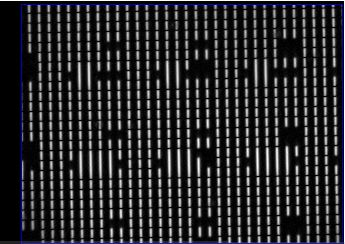Figure: Horizontal Derivative Image, Absolute Value.

Notice how both the left and right edges of each square appear as white lines in the derivative image. A dark-to-light transition has the same appearance in the absolute-value derivative as a light-to-dark transition. It is this absolute value that allows us to find the edges in the chessboard pattern, because in the absolute-value derivative, the edges are continuous and bright.

Source Code: The routines for calculating derivative images are image_grad_i and image_grad_j, defined in image_manip.pas. The image structure itself is defined in images.pas.

## Fourier Transform

We take a strip along the top of the horizontal derivative image and calculate its vertical intensity profile by summing the intensity of the pixels in each of its columns. We calculate the spatial frequency spectrum of this profile using a discrete fourier transform. To improve the performance of the fourier transform, we first subject the vertical intensity profile to a window function. When our original image contains a chessboard pattern, the largest component in the spectrum will correspond to the spatial frequency of the chessboard squares. The phase of this component corresponds to the position of the first chessboard edge from the left side of the image.

Source Code: Fourier analysis of intensity-profiles takes place in rasnik_find_pattern, which calls profile_by_fourier. The profile_by_fourier routine makes a single call to fft_real, which is defined in utils.pas.

Our fast fourier transform insists upon an exact power of two number of samples. If we have 320 points in our profile, we pass to the fast fourier transform these 320 points along with another 192 points whose value we set equal to the average value of the first 320. These 512 points give us 256 discrete frequency components in the transform. Each frequency is a multiple of the fundamental frequency represented by a strip 512 columnes wide. The first term in the spectrum is the zero frequency term, or average intensity. The second term has period 512 columns. The last has period two columns.

Example: Suppose we have squares that are roughly four pixels high, and we use 256 rows for our fourier transform. Our spectrum contains components with the following periods close to four pixels: 3.46, 3.51, 3.56, 3.66, 3.71, 3.76, 3.82, 3.88, 3.94, 4.00, 4.06, 4.13, 4.20, 4.27, 4.34, 4.41. The estimate we obtain from our fourier transform will be within 1% of the correct period.

Here is the fourier spectrum we obtain for a strip 30 pixels high along the top of the horizontal derivative of three of our sample images. We plot the amplitude of the components versus the period in pixels, as opposed to versus frequency in 1/pixels. You will find these images in our LWDAQ/Images directory.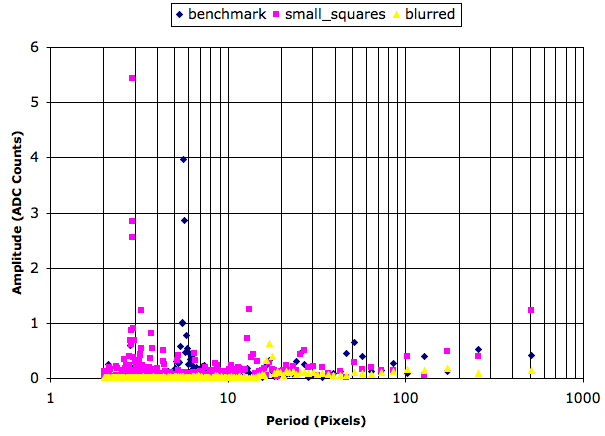Figure: Discrete Fourier Transform of Horizontal Slice. The slice is 30 pixels high, taken along the top of the horizontal derivative of three different rasnik images.

As you can see, each spectrum has a peak intensity that stands out from the rest of the spectrum. If we consider the ratio of the peak amplitude to the average amplitude, we obtain a simple criteria for rejecting images that contain no periodic pattern. The peak ratio for a sharp rasnik image is between 20 and 40. For a blurred image with nine squares across, the ratio is still 10. When we reduce the exposure time until we can barely see any sign of the pattern, even with image intensification, the peak ratio drops to 3.0. Noisy or blank images, however, have peak ratios less than 2.0. We choose 3.0 as our threshold for acceptance.

Source Code: The rejection is set by min_peak_ratio in the profile_by_fourier routine for LWDAQ 7.5 and later.

The following image has peak to average ratio of 5.0. It is Rasnik_dim from our LWDAQ/Image directory. We use exact intensification in the Rasnik Instrument so you can see the rasnik pattern, which would otherwise be invisible. We acquired Rasnik_dim from our demonstration stand using exposure time 200 μs.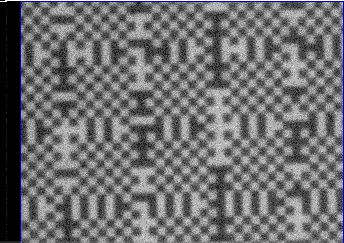Figure: A Dim Rasnik Image. This is Rasnik_dim from our sample collection. Standard deviation of image intensity is 0.5 ADC counts.

The period and phase of the largest component in the spectrum are a good estimate of the size and offset of the chessboard pattern. But the discrete jumps from one frequency to the next in the spectrum prevent us from obtaining a more accurate measure of the period with the fourier transform. If possible, therefore, we try to refine our measurement of period and offset by taking a closer look at the profile, knowing ahead of time the approximate size of the squares.

## Smoothed Peaks

The fourier analysis of our gradient image gives us the spatial period of the mask pattern to within 1% when the square are 4 pixels across. If we have 80 squares across our image, this 1% error in spatial frequency will cause our estimate of the locations of the peripheral pattern edges to be misplaced by up to 1% * 80 / 2 = 40% of a square width. That's not good enough. Our objective in analyzing the image slices is to isolate one edge from another so that we can fit lines to the edge pixels and obtain the edge locations with as much accuracy as possible. Before we can do that, we need to be sure that we know where the edges are to within at least 10% of a square width.

To improve our measurement of square size and offset we use a method of smoothed peaks. Using approximate knwoledge of the square size, we create a spatial band-pass filter and apply it to our intensity profile. We do this for every slice down to the bottom of the image. In each slice, we try to determine the square size from the locations of the maxima in the filtered profile.

Source Code: The number of slices we analyse in each direction on the image is given by rasnik_num_slices. The more slices, the greater the mask rotation we can accommodate. The smoothed peak routine is profile_by_maxima, called from rasnik_find_pattern, both in rasnik.pas. Starting in LWDAQ 7.5, whenever the filtered profile fails to give us a good estimate of the period and offset, we revert to the estimate provided by a fast fourier transform of the slice.

The spatial band-pass filter gives us peaks that correspond to edges in the pattern. Some edges do not appear as maxima because the edges do not appear in the slice. The following image shows the horizontal band-pass filtered profiles for eight slices.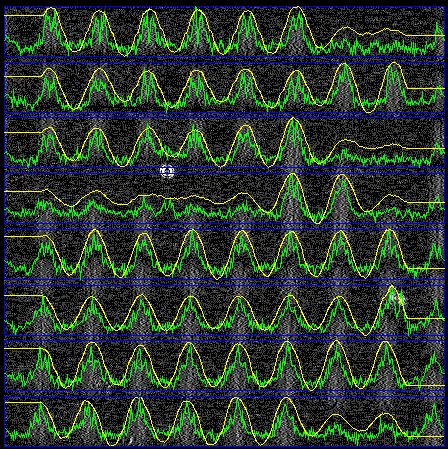Figure: Band-Passed Filtered Profiles in Slices. The unfiltered profiles are green. The filtered profiles are yellow. Both are plotted over the horizontal gradient image. The blue boxes outline the slices. We obtain this plot with show_fitting set to −1 in the Rasnik Instrument. The image is Rasnik_large.

In some slices we see that peaks are missing or obscured. Code squares disrupt the regularity of the chessboard in the slices. Nevertheless, we almost always overcome these irregularities, and we use the peaks to obtain a better estimate of the pattern frequency, rotation, and offset. We call the resulting estimate our approximate pattern. If it so happens that one of the slices fails to provide a useful sequence of maxima, we calculate the fourier transform of the profile and use the period and phase of the largest component instead. In that case, the filtered profile will be red in a plot like the one we show above.

## Line Fitting

Our approximate pattern is good to a few microns in position, but only 1000 ppm in magnification. We would like our magnification accuracy to approach 10 ppm. So we go on to refine the fit further. The approximate pattern is good enough to allow us to isolate edges in the derivative images. Given any bright pixel in the derivative image, we can tell which edge it corresponds to, and add it to a straight-line fit that will tell us with better accuracy where the edge is.

We divide the derivative image into strips parallel to the vertical edges, and fit straight lines to the pixels in each strip. We weight each pixel by its intensity so as to favor brighter edge pixels. Nevertheless, noise pixels far from the line can displace the fit significantly. We consider the slope and offset of all the lines and reject any that are more than two standard deviations from the mean obtained from the entire set.

Source Code: The straight-line fitting is done in rasnik_refine_pattern, which takes as input not only the horizontal and vertical derivative images, but also the approximate pattern obtained by rasnik_find_pattern.

The following figure shows the lines we fitted and used in our analysis of a sharp rasnik image. We see that even in a good image, we reject some of the lines, and these tend to be ones most distrupted by dirt or code squares.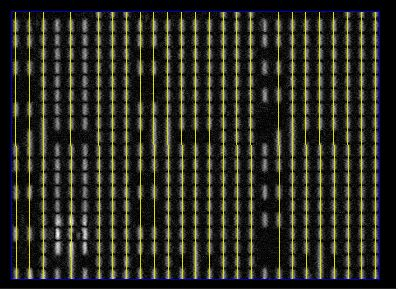Figure: Lines Fitted to Derivative Image Pixels.

When the magnification of the mask changes with position in the image, we say the image has skew. The change in magnification from left to right is the x-direction skew, and from top to bottom is the y-direction skew. We measure the x-skew by looking at how the slope of the horizontal lines changes from top to bottom in the image. We express the skew in units of mrad/mm. When the x-skew is positive, the horizontal lines are diverging from left to right. The y-skew we obtain from the variation in the slope of the vertical lines from left to right. When the y-skew is positive, the vertical lines are diverging from top to bottom. In a skewed image, the pattern x and y-directions are not everywhere perpendicular in the image. If they are perpendicular at the center of the analysis bounds, where our analysis places the origin of the pattern coordinate system, then the x and y skew parameters are sufficient to describe the skewed image, including correct orientation of the pattern coordinates in other parts of the image where they are not perpendicular. But if the pattern coordinates are not perpendicular at the center of the analysis bounds, we must account for this non-perpendicularity with another rasnik parameter: the image slant. The slant is the angle by which the pattern coordinates are less than perpendicular at the pattern origin.

## Code Squares

Now that we have the frequency, rotation, and offset of the chessboard-like mask pattern, we need to set the parity of the pattern, which is to say: we need to know which squares are nominally white, and which are nominally black. Code squares are squares that are out of parity. The critical operation in determining the parity of the pattern, and then the parity of individual squares with respect to the pattern, is determining whether or not each square is black or white. Because the intensity of the mask illumination can vary across the mask, we cannot rely upon comparing each square to an average intensity. Nor can we take a single pixel from a square and compare it to a single pixel in neighboring squares. Dust and noise in the image will defeat any judgment that uses single pixels.

Source Code: The square_whiteness routine is in rasnik.pas returns the whiteness of a square. rasnik_identify_code_squares decides which squares are code squares and which are not, by comparing its center intensity to the center intensity of its neighbors. We calculate the center intensity of each square in one pass through the pattern array with rasnik_identify_pattern_squares.

We do, however, need some measure of what is a large variation in intensity in the rasnik image, in order to determine if a variation between one square and the next is significant. So we use the standard deviation of intensity as our measure of significant intensity variation. We compare the amount by which a square is whiter than its neighbors to the standard deviation of the image intensity, and so decide if the difference is significant.

Source Code: The image_amplitude routine is in utils.pas. It is not deterministic: it determines the amplitude of an image by looking at 10000 random points in the image, and is several times faster than a routine that looks at all points in the image. You can see this non-determinism sometimes, when a dim image has a piece of dirt that almost turns a white square into a black square. Sometimes the routine decides the square is a code square, and sometimes not. This is because of tiny variations in the result of image_amplitude.

Identifying the code squares requires that we determine the center intensity of each square, and compare it to the center intensity of its neighbors.

Once we identify the code squares, we try to interpret them. This endeavor is greatly complicated by the fact that there may be many false code squares in the image, and by the fact that we may not know the orientation of the mask pattern. On the other hand, correct code squares are severely constrained in the way they relate to one another. By checking the available code squares against the constraints of an uncorrupted rasnik pattern, we can reject erroneous code squares, determine the correct rotation of the mask, and so arrive at an accurate and reliable measurement.

Source Code: The code analysis is based in rasnik_analyze_code, in rasnik.pas. This routine calls analyze_orientation, in which you will find the bluk of the code-square interpretation and checking.

The analyze_orientation routine assumes a particular mask orientation and goes through all the canditate pivot squares in the image calculating their x- and y-direction code values from adjacent code squares. It then looks for sets of pivot squares whose x- and y-codes agree. It assigns a score to the orientation, which is the number of pivot squares in the largest set of pivot squares that agree with one another.

In dim and dirty images, we can get a large number of candidate pivot squares, and these can form several mutually-agreeing sets of the same size. We reject an orientation under such circumstances, by setting the orientation score to zero. We check to see if the number of acceptable pivot squares is above a certain fraction of the available pivot squares. In this way, we reject an orientation if there are too many unacceptable pivot squares. We also check to see if all acceptable pivot squares are separated by an integer multiple of code_line_spacing squares in both the x- and y-directions.

## Display

When it's finished anlyzing the code squares, the analysis routine draws its results on top of the image, as you can see here. It marks pattern edges with green lines and code squares with little rectangles. The rectangles are red if the code square lies at the intersection of two valid binary code numbers. We call the red squares pivot squares. The rectangles are yellow if the code square is not a pivot square. If you have show_fitting set when you call the analysis, you will see all the remaining, usable squares in the image marked with a blue rectangle. The display makes it easy for us to identify the cause of analysis failure, and has been of great help to us in our efforts to improve and accelerate the analysis.

## Blank Images

When we pass a non-rasnik image to the analysis, we want to get an error message as a result, not a rasnik measurement. Starting with LWDAQ 7.5, we use the fourier transform stage of analysis to reject blank or exceedingly distorted rasnik image. Earlier versions of the code relied upon examination of the code squares. These earlier versions would occasionally produce a result like the one shown below.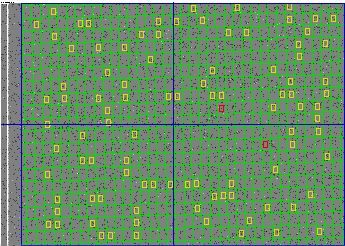Figure: False Positive on a Blank Image Obtained with LWDAQ 7.3. We have not yet observed a false positive with LWDAQ 7.5.

When we obtain an apparantly valid rasnik measurement from a non-rasnik image, we call it a false positive. The false positive rate with noise images like the one shown above is less than 0.01% for LWDAQ 7.5 and roughly 0.02% for LWDAQ 7.4 and earlier. The greatest advantage of LWDAQ 7.5 is the rapidity with which it rejects non-rasnik images. The rejection occurs before pattern refinement and code analysis, and therefore takes a quarter as much time as a complete analysis. This rapid rejection of invalid images greatly accelerates our use of random boundries, which we describe in the next section.

## Random Boundaries

When a significant portion of a rasnik image is obscured or distorted by obsticles or dirt, we must find analysis boundaries that enclose the intact portion of the image before we can expect successful analysis. The Rasnik Instrument in our LWDAQ Software will select suitable analysis boundaries automatically after analysis failure if we instruct it to do so. Our Pascal libraries do not perform this selection. Instead, we implement a simple random try-out algorithm in the Tcl. The LWDAQ_analysis_Rasnik routine generates analysis boundaries at random and applies rasnik analysis to the image. It continues trying random boundaries until the analysis succeeds or it reaches its maximum permitted number of attempts. We describe how to set up the random analysis boundary selection in the Rasnik Instrument section of the LWDAQ User Manual.

When we use random boundaries, we will lose measurement accuracy because we are not using all available squares. If we are using the center of the image sensor as a reference point, different analysis boundaries give different mask rotations, and these rotations are multiplied by the distance from the analysis boundary center to the image sensor center when we calculate the point in the mask that is projected onto our reference point.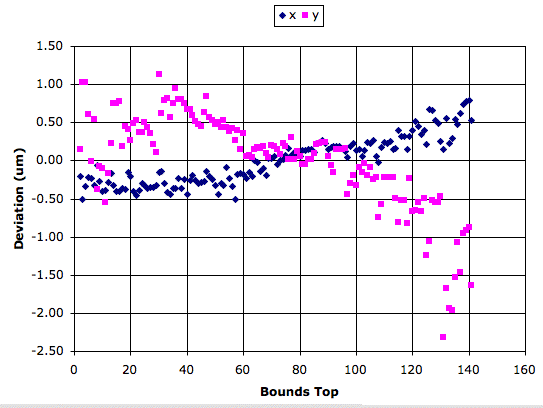Figure: Variation in x and y with Changing Boundaries. We use an area one hundred rows high, spanning the width of the image. The reference point for the rasnik measurement is image center.

The graph above shows how x and y position vary as we use a 100-pixel high subset of the analysis bounds drawn on one of our example images, Rasnik_benchmark. We obtained this image with the thin-lense camera mounted on our LWDAQ Demonstration Stand. You will find it in our LWDAQ distribution, in the Images folder. We move our subset down from the top of the right side of this image to the bottom. At each step, our bounds cover the full width of the image, but less than half the height. The change in x as we move our bounds downwards is less than half the change in y.

We obtained the above results with the following Toolmaker script, which you can use yourself to obtain similar plots from your own images. Cut and paste the above code into the Toolmaker window and press Execute.

```set fn [LWDAQ_get_file_name]
set c [lwdaq_image_characteristics \$r]
scan \$c %u%u%u%u left top right bottom
set height 100
for {set a \$top} {\$a <= [expr \$bottom - \$height]} {incr a} {
lwdaq_image_manipulate \$r none -left \$left -right \$right \
-top \$a -bottom [expr \$a + \$height]
set result [lwdaq_rasnik \$r \
-square_size_um 120 \
-pixel_size_um 10 \
-reference_x_um 1720.0 \
-reference_y_um 1220.0 ]
LWDAQ_print \$t "\$a \$result"
LWDAQ_support
}```

[19-MAR-10] We applied the same analysis to an image sento us by NIKHEF and obtained this graph, in which we see a 15-μm change in x as we move down the image. Thomas Bauer is looking into whether it is the image that contains the errors, or whether the analysis creates them.

## Pre-Filtering

When analysis fails on a dim image, it often does so because quantization noise in the image is dominating the intensity-gradient of the rasnik pattern. If the squares of the pattern are large, we can filter the image before analysis so as to attenuate the quantization noise. The smooth manipulation provided by our lwdaq_image_manipulate library routine applies a 3×3 box averaging filter to the original rasnik image, and analyzes the filtered image. The smoothed image is not only filtered, but also intensified. The final image always has intensity black (0) to white (255). The intensification means that we greatly attenuate quantization noise in the filtered image.

The Rasnik Instrument of our LWDAQ Software allows you to smooth the image before analysis by setting the value of its analysis_enable parameter.

If the squares in our image are large, we can shrink the image by a factor of two and still find the pattern. Indeed, we can sometimes shrink the image by a factor of four and still find the pattern. The Rasnik Instrument allows us to smooth and shrink before analysis through its analysis_enable parameter. The table below gives the rasnik measurement we obtain from an with large squares, with a various combinations of pre-filtering.

Pre-FilteringResultTime (ms)
none 9785.80 32991.31 4.277918 4.271931 -30.422 0.326 85.0 7.4 250
double-smooth 9786.24 32991.19 4.285319 4.280173 -27.115 0.233 85.0 7.4 250
single-smooth, shrink ×2 9786.22 32991.24 4.282743 4.281735 -26.977 0.217 85.0 14.8 80
single-smooth, shrink ×3 9786.26 32989.54 4.281528 4.283042 -27.086 0.144 85.0 22.2 50
single-smooth, shrink ×4 9784.58 32991.40 4.282412 4.283225 -27.123 0.106 85.0 29.6 40
Table: Rasnik Analysis Results for Various Pre-Filters. We used our Rasnik_large library image. The result values are x (μm), y(μm), x_magnification, y_magnification, rotation (mrad), precision (μm), square size (μm), pixel size (μm). The analysis time does not include the time taken to pre-filter the image. Pre-filtering takes around 100 ms for the Rasnik_large image.

[17-MAR-11] The x and y measurements vary by less than 2μm as we smooth and shrink the image. The magnification varies by around 0.2%. Even smoothing the image changes the magnification by 0.2%. The Rasnik_large image does not have sharp edges, so we do not expect the magnification measurement to be accurate. But we see that shrinking the image does not make things significantly worse.

## Measurement Precision

Our rasnik analysis produces an estimate of its own measurement precision. The error field in our result, which we call the mask error, is the uncertainty in the x and y fields. A rasnik measurement gives us the point in the mask that is projected onto a reference point in the image sensor. The mask error applies to the measured point in the mask, not to the reference point in the image. Nevertheless, our choice of reference point affects the mask error.

Our Rasnik Instrument supports several standard reference points and also custom reference points through its analysis_reference_code, analysis_reference_x_um, and analysis_reference_x_um parameters. The most accurate reference point is the center of the analysis bounds. But this point is not practical in conjunction with random boundaries.

The reference code parameter tells the Rasnik Instrument how to choose the reference point for analysis. The analysis result will be the coordinates of the point in the mask that is projected onto the reference point. Here are the values of reference code defined in Rasnik.tcl.

```rasnik_reference_top_left_corner=0;
rasnik_reference_center_analysis_bounds=1;
rasnik_reference_center_ccd=2;
rasnik_reference_image_point=3;```

The reference point is a point in image coordinates, and is given in units of micrometers. The image coordinate origin is in the top-left corner of the image. The x-axis runs left to right and the y-axis runs top to bottom. If we use reference code 3, the Rasnik Instrument will use reference_x_um and reference_y_um to specify the reference point. The other reference codes tell the Rasnik Instrument to ignore reference_x_um and reference_y_um, and instead calculate the reference point itself. In the ATLAS end-cap we use the center of the image sensor as our reference point with reference code 2. When we compare different rasnik analysis routines, we use the top-left corner of the top-left pixel with reference code 0.

The center of the images we obtain from our TC255P sensors in the ATLAS end-cap alignment system is (1720 μm, 1220 μm) in image coordinates. For the ICX424 and ICX424Q the center is (2590 μm, 1924 μm).

To calculate the mask error, we start with our estimate of the error in measuring the position of the rasnik pattern at the center of the analysis bounds in the image. We obtain this estimate by looking at the standard deviation of the residuals from the multiple straight-line fits we perform to refine our rasnik pattern. We divide the standard deviation of the residuals by the square root of the number of edge pixels we used. We call this error at the center of the analysis bounds on the image the center point error. We estimate the rotation error by taking the center point error and dividing by the width of the analysis bounds. We multiply the rotation error by the distance from the center of the analysis bounds to the reference point, wherever it may be. This gives us an additional error that we add in quadrature to the center point error to obtain our estimate of the error at the reference point.

Er = EcEcr/w = Ec(1 ⊕ r/w),

where Er is the reference point error, Ec is the center point error, r is the distance from the center of the analysis bounds to the reference point, w is the width of the analysis bounds, and ⊕ is the act of adding in quadrature. We divide the reference point error by the magnification to obtain the mask point error.

As we mentioned above, we estimate our rotation error by dividing the center point error by the width of the analysis bounds (Ec/w). But our rasnik analysis provides us with only the mask error. We can convert the mask error into the reference point error by multiplying by the magnification. We could use the above equation to calculate the center point error from the reference point error, but doing so would require that we knew w and r, the width and center of the analysis bounds with which the rasnik analysis was performed. In general, we don't know these things when we look up an old rasnik result.

We recommend that you estimate rotation and magnification error by dividing the reference point error by the image width. This calculation won't be far wrong when you have analysis bounds that take up more than half the image, but for smaller boundaries, you will under-estimate the error.

Example: We analyze Rasnik_small_bounds, which uses a small analysis boundary in the lower-right of the image. We obtain a mask error 0.8 μm at the center of the analysis bounds (2965 μm, 1965 μm), 1.2 μm at the center of the image (1720 μm, 1220 μm), and 2.3 μm at the top-left corner of the image (0 μm, 0 μm). The magnification is 0.8, so our reference point error in these three cases is 0.64 μm, 1 μm, and 1.8 μm respectively. If we divide by the image width of 3400 μm, we obtain rotation errors 230 μrad, 300 μrad, and 680 μrad. The magnification error will be similar.

As you can see in the example, our rule of thumb leads to differing rotation and magnification errors for the different reference points. But the choice of reference point should not affect either error estimate. Only the position error is affected by reference point. But when we use larger boundaries, our rule of thumb works rather better.

Example: We analyze Rasnik_small_bounds, but with bounds that fill the entire image. We obtain a mask errors 0.09 μm, 0.09 μm, and 0.10 μm respectively. When we divide by the image width we get rotation error 30 μrad and magnification error 30 ppm.

The best images give us a magnification uncertainty of around 30 ppm. When we analyze simulated images, our magnification precision can be as low as 10 ppm, but we have systematic errors much larger than our precision, as we describe in Simulated Images.

## Simulated Images

One way to test our rasnik anslysis is with simulated rasnik images whose position, rotation, and magnification we know exactly. In rasnik.pas we define a procedure called rasnik_simulated_image. This procedure draws a rasnik pattern in an image. We call this routine from our LWDAQ command line with lwdaq_image_manipulate with the rasnik manipulation. We can specify the location of the top-left corner of a black square in image coordinates, the width and height of the squares in pattern coordinates, the rotation of the pattern, and the sharpness of its intensity variation. The simulated images contain no code squares.

[10-FEB-11] We test the analysis of LWDAQ 7.5.8 with simulated images. We used the following Tcl script in our Toolmaker to generate images with rotation −150 mrad to 150 mrad in steps of 10 mrad. Each image has white intensity noise added, of peak-to-peak amplitude one count.

```lwdaq_image_create -width 400 -height 400 -name sim
catch {image delete p}
image create photo p
destroy \$f.i
label \$f.i -image p
pack \$f.i
for {set rot -150.0} {\$rot <= +150.0} {set rot [expr \$rot + 10.0]} {
LWDAQ_print -nonewline \$t "[format %.1f \$rot] "
foreach s {0.02 0.1 1.0 10.0} {
lwdaq_image_manipulate sim rasnik "200.0 200.0 20.0 20.0 \$rot \$s 1.0"
set result [lwdaq_rasnik sim -pattern_only 1]
LWDAQ_print -nonewline \$t "[format %.6f [lindex \$result 2]] "
lwdaq_draw sim p -intensify exact
LWDAQ_update
if {![winfo exists \$t]} {break}
}
LWDAQ_print \$t
}```

We place the top-left corner of a black square at location (200, 200) in image coordinates, which places the square 200 pixels to the right of the top-left corner of the top-left pixel and 200 pixels below. The squares are 20 pixels wide and 20 pixels high. We vary rotation from −150 mrad to +150 mrad. We vary sharpness from 0.02 to 10. We display these images with our exact intensification, which means that the brightest pixel appears white and the dimmest pixel appears black. At sharpness=0.02 the image intensity is sinusoidal with amplitude 2% of full scale. At sharpness=0.1 the amplitude is 10% and at sharpness=1 the amplitude is 100%. These two images look the same with exact intensification. At sharpness=10, the sinusoid is amplified by 10 and clipped to the black and white levels to give a near-perfect chessboard pattern.

Note: Analysis fails on images with sharpness=0.01 or less. Quantization is a non-linear process that creates a double-frequency signal. In the derivative of an image with peak-to-peak amplitude 2 counts, the double-frequency component is larger than the fundamental, as you can see here.

We apply partial rasnik analysis using the -pattern_only option in lwdaq_rasnik. We measure the location and square size of the pattern. The following graph shows how our magnification varies with rotation for the four different values of sharpness.Figure: Magnification Error versus Rotation for Various Values of Sharpness. The squares are 20 pixels wide in an image that is 400×400 pixels.

Our magnification resolution is roughly 200 ppm for rotations ±100 mrad, but degrades rapidly for greater angles. We obtained plots in the same format for the measured position and rotation. Our position accuracy over the full range of rotation and sharpness is better than 1% of a pixel. Our rotation resolution is around 50 μrad for sharpness greater than 0.01.

[08-FEB-11] We use the simulated images to test the x-direction measurement of the LWDAQ 7.5.8 analysis. Instead of rotating the images we displace them in 0.02-pixel steps and compare the simulated image position with the measured position. We use the following Toolmaker script to produce the graph that follows.

```lwdaq_image_create -width 400 -height 400 -name sim
catch {image delete p}
image create photo p
destroy \$f.i
label \$f.i -image p
pack \$f.i
for {set x 202.0} {\$x <= 207.0} {set x [expr \$x + 0.02]} {
LWDAQ_print -nonewline \$t "[format %.2f [expr fmod(\$x,10)]] "
foreach s {0.02 0.1 1.0 10.0} {
lwdaq_image_manipulate sim rasnik "\$x 200.0 20.0 20.0 0.0 \$s 1.0"
set result [lwdaq_rasnik sim -pattern_only 1]
set rx [expr fmod([lindex \$result 0],10)]
LWDAQ_print -nonewline \$t "[format %.2f \$rx] "
lwdaq_draw sim p -intensify exact
LWDAQ_update
if {![winfo exists \$t]} {break}
}
LWDAQ_print \$t
}```

Our image simulation provides for the inclusion of white noise and we add noise of peak-to-peak amplitude one count to all our images. Thus the images are more like those we obtain from our cameras. We obtain the following graphs of the measurement error for our four values of sharpness. The graph shows systematic errors with period one pixel that get less severe as the image becomes sharper.Figure: Position Error versus Position for Various Values of Sharpness. The squares are 20 pixels wide in an image that is 400×300 pixels. The simulated images include noise of peak-to-peak amplitude one ADC count. We obtained these results with LWDAQ 7.5.8.

The average position error is −0.038, 0.000, 0.000, and 0.000 pixels with increasing sharpness. The standard deviation of the error is 0.17, 0.09, 0.07, and 0.01 pixels with increasing sharpeness.

[10-DEC-08] We receive a report from Rikard Sandstrom at CERN that our magnification measurements are in error when the mask is rotated. Harry van der Graaf of NIKHEF produced a library of simulated images with rotation from -100 mrad to +100 mrad. Unlike our own simulated images, these images contain code square markings. You will find them in NIKHEF_Images.zip. Rikard sent us a graph of our magnification error versus image number and compared to the magnification error from the FOAM and FORTRAN rasnik analysis programs. It turns out that we broke our magnification calculation back in late 2007. Instead of multiplying by cos(rotation) in several places, we were dividing by cos(rotation). We fixed the bug and Rikard obtained another graph in which the error looks similar to the one we obtain ourselves.

We analyzed the NIKHEF images ourselves with LWDAQ 7.3.20 with the following Tcl script, which we executed in our Toolmaker.

```upvar #0 LWDAQ_config_Rasnik iconfig
upvar #0 LWDAQ_info_Rasnik iinfo
set iconfig(analysis_enable) 3
set iconfig(analysis_reference_code) 3
set iinfo(analysis_min_width) 250
set iconfig(image_source) file
LWDAQ_open Rasnik
set d [LWDAQ_get_dir_name]
set fl [glob -nocomplain [file join \$d *.gif]]
set fl [lsort -dictionary \$fl]
foreach  f \$fl {
set LWDAQ_config_Rasnik(file_name) \$f
set result [LWDAQ_acquire Rasnik]
LWDAQ_print \$t \$result
LWDAQ_support
if {![winfo exists \$t]} {break}
}```

We obtained the following graph of magnification and position deviation with rotation.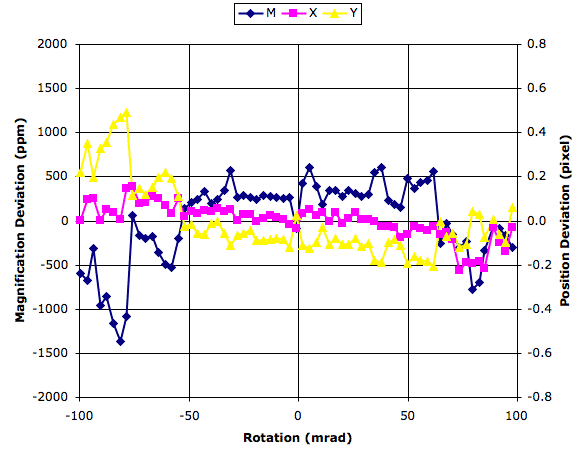Figure: Deviations in Magnification and Position versus Rotation in NIKHEF Images. We do not know the exact rotation values associated with each image. We assumed that the mask was rotating about the top-left corner of the image. In order to make our measurements independent of mask square size, we convert the position measurement from microns in the mask to pixels in the image sensor. The pixels in our sensors are 10 μm square, so a 0.1 pixel deviation is 1 μm.

The rotation in these patterns appears to take place about the top-left corner of the image instead of the center. Our position measurement is therefore coupled to our rotation measurement. It may be this coupling that gives us inferior position results compared to those we obtained with our own simulated images. Our own images rotate about the center of the image.

## Analysis Failure

The following constants in our code put limits on the square patterns it can analyze successfully. For a throughh explanation of the function of each constant, see rasnik.pas. These constants can be altered only by editing the source code and re-compiling. We summarize their functions below.

```rasnik_min_squares_across=8; {squares}
rasnik_max_squares_across=200; {squares}
rasnik_min_pixels_per_square=2.5; {pixels}
rasnik_num_slices=8;```

The analysis will fail on any image in which there are fewer than rasnik_min_squares_across from left to right or from top to bottom of the analysis bounds. It will fail if there are more than rasnik_max_squares_across squares from left to right or top to bottom. It will fail if the squares are less than rasnik_min_pixels_per_square pixels wide or high.

If we reduce the minimum square size to two pixels, we find that the analysis is fooled by blank or dark images. A two-pixel square gives a two-pixel periodic pattern in the derivative image. It is not possible for us to obtain the phase of such a pattern with accuracy better than 180°, so there is not point in attempting to analyze such a pattern. With a two-pixel minimum size, our analysis is fooled by our BCAM_250cm sample image into seeing an array of 100×100 two-pixel squares, resulting in a futile ten-second attempt to find a pivot square.

Note: Up until 7.2 the minimum square size was 4.0. In 7.2 we reduced it to 3.0 pixels to accommodate some images sent to us by Harry van der Graaf. In 7.3 we reduced the square size to 2.0 pixels. In 7.5, we increased it to 2.5 pixels after observing problems with our sample images.

The rasnik_num_slices constant tells us how many slices the analysis takes across the image in order to obtain the pattern rotation. With eight slices, the routine divides the image from top to bottom into eight equal slices, each of them as wide as the analysis bounds. Each slice provides square edge locations. So long as the edges in adjacent slices line up to within less than half a square width, the pattern rotation will be unambiguous to the routine. Thus rasnik_num_slices determines the maximum acceptaebl rotation of the pattern, which is half a square per slice. With eight slices, the pattern can shift by four squares from top to bottom and four squares from right to left. Any additional rotation will result in analysis failure.

Analysis will fail on blank images, or upon images that are so blurred and noisy that the fourier analysis of slices cannot determine the mask pitch.

Even if the analysis finds the chessboard pattern, it might still fail because it cannot understand the image code squares. Dirt on white squares makes them look black, so they appear to be code squares. If there is dirt on the actual code lines in the image, the analysis may not be able to find a good pivot square.

## Skewed Images

[22-DEC-17] The following image is our example Rasnik_skewed image with the results of analysis overlayed. The x-direction skew is 4.0 mrad/mm, and the y-direction skew is 0.1 mrad/mm. We can see the squares getting bigger in the x-direction. We can also see that the squares are taller than they are wide. Both effects are due to the mask being rotated about the vertical axis with respect to the image sensor.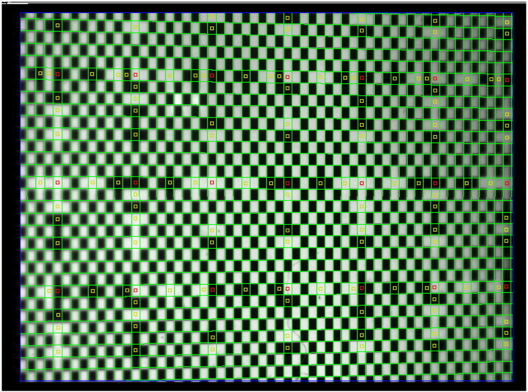Figure: A Skewed Rasnik Image. The mask is rotated about the vertical axis with respect to the image sensor.

Image skew is caused by one part of the mask being nearer to the lens than the other. In the example above, we have rotated the mask about a vertical axis within the plane of the mask itself, so that the right side is farther away then the left. When the mask is one meter from the lens, the only effect of such rotation is to change the relative sizes of the mask squares in the vertical and horizontal directions. But when the mask is 10 cm from the lens, the skew causes a visible change in square size across the image, and a visible divergence of the mask edges as well.Figure: Image Skew for Mask Rotated by 45° About Mask Y-Axis. The image distance is 91 mm. We expect the skew to be be tan(45°)/91 mm, or 11 mrad/mm for 45°, which agrees well with our observations.

The x-direction skew is a function of the rotation of the mask about the mask y-axis and the distance from the lens to the image sensor. According to our calculations, we have Sx = tan(α)/b, where α is the rotation and d is the image distance. We also expect α = acos(mx/my), where mx and my are the x and y magnification.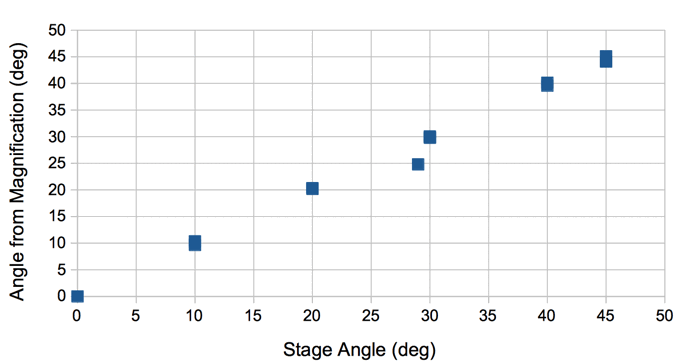Figure: Mask Rotation About Y-Axis Measured By Magnification Ratio versus Stage Rotation.

The following derivation shows how image coordinates relate to mask coordinates. We use the suffix p for mask coordinates, because these same calculations apply to any object with a regular pattern of edges. Here we are simplifying our calculation by assuming that the mask is rotated about its y-axis only.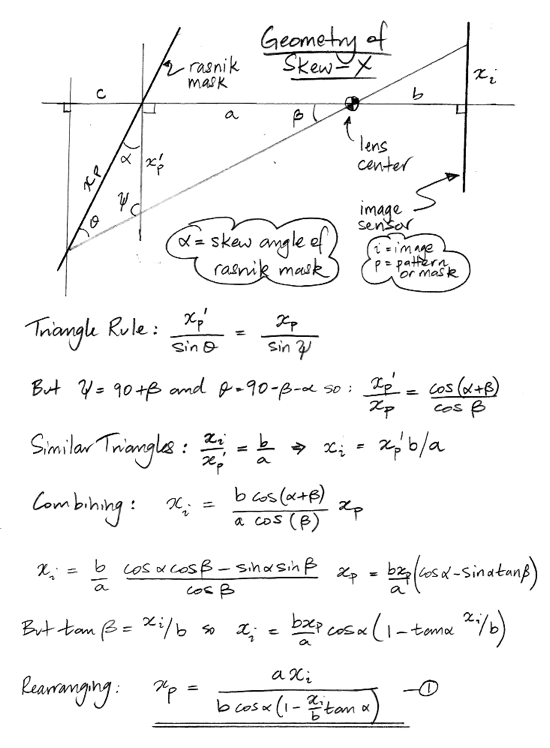We use the above equation, the assumption that the pattern edges are perpendicular in the image at the pattern origin, as well as the approximation b >> xi to arrive at an expression for the image skew returned by the rasnik analysis.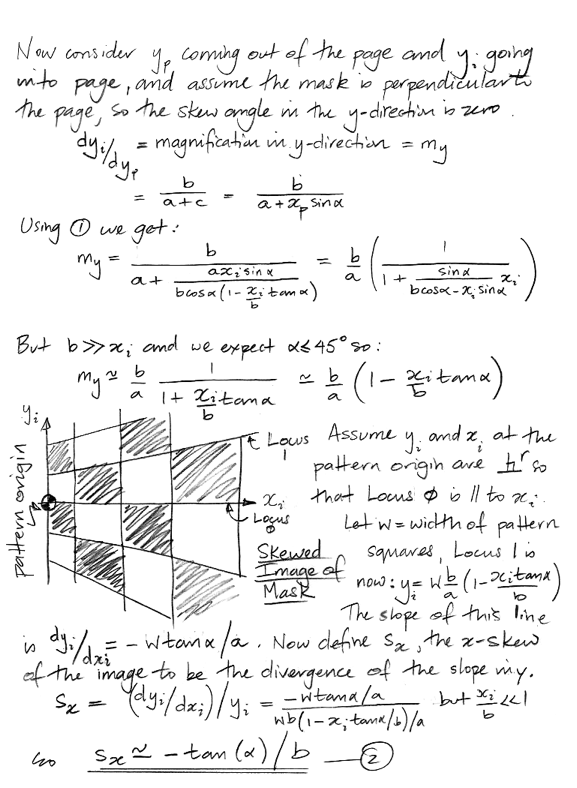The skew appears in all coordinate transformations between the pattern and image. We derive the transformation equations for rotation of the mask only about its own y-axis below.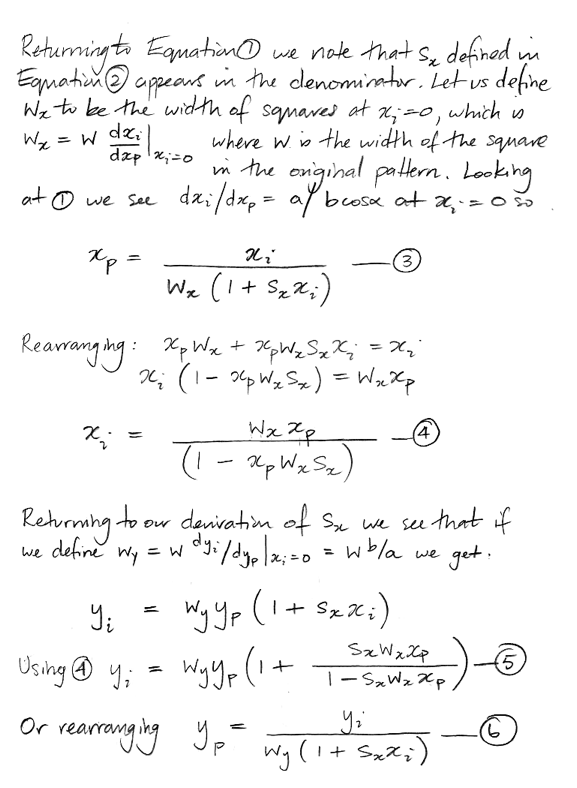The x and y skew parameters are sufficient to describe the mask image provided the pattern edges are perpendicular at the center of the analysis bounds. In general, however, the pattern edges will not be perpendicular in the image at the pattern origin. The slant of the pattern is the angle by which the pattern x and y directions are less than perpendicular in the image.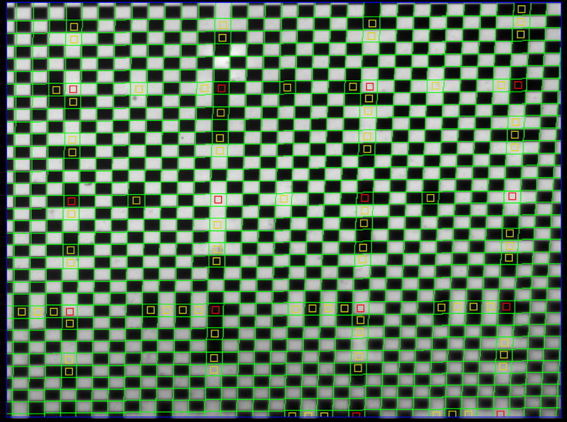Figure: A Skewed and Slanted Rasnik Image. The mask is rotated about the horizontal axis with respect to the image sensor, and is such a way that the pattern coordinates are not perpendicular at the center of the image.

The image above has slant −39.13 mrad, x-skew −0.00 mrad/mm, and y-skew −9.83 mrad/mm. The rotation is −11.34 mrad, which is the average rotation of the pattern coordinates at the center of the analysis boundaries. In this case, we see that the angle between the pattern coordinates is 39.13 mrad greater than perpendicular, while the pattern diagonal line is rotated by 11.34 mrad clockwise in the image. Assuming we have corrected for skew and rotation already, the correction for slant will be a linear transformation as derived below.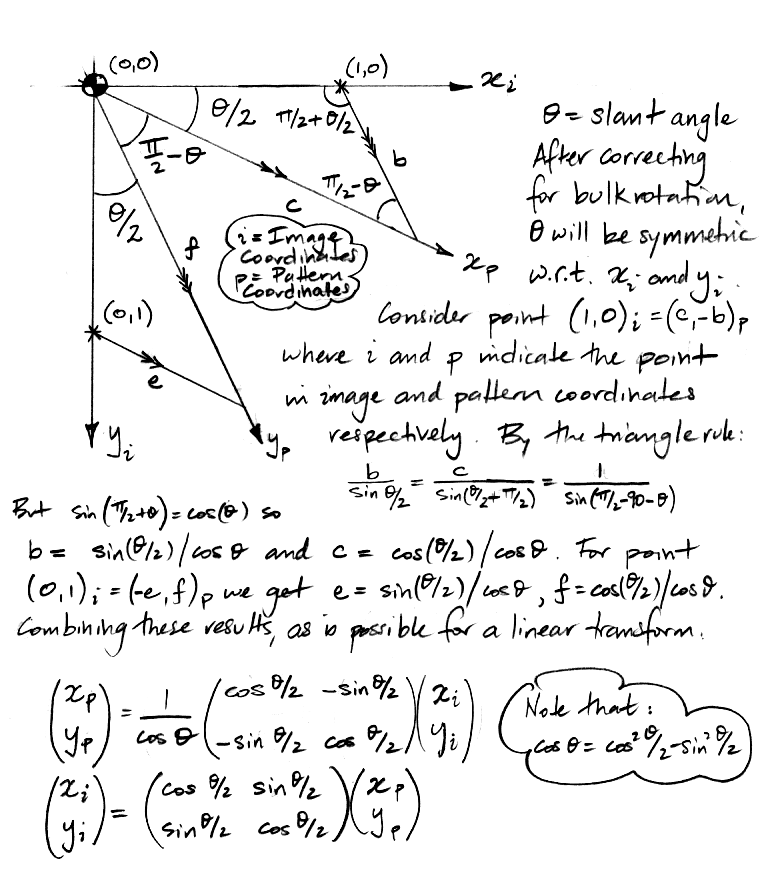Image slant can occur even when there is no significant image skew. When we rotate the mask about an axis parallel to its surface but not parallel to either of its coordinate directions, the squares of the mask will appear slanted, regardless of whether or not there is a change in magnification from one side of the image to the other. The squares are being stretched in a direction that is parallel to neither of their edges, and so they become diamond-shaped.Figure: A Slanted Rasnik Image with No Skew. The mask is rotated about an axis in its plane that is not parallel to either of its own coordinates. The mask is far enough from the lens that the change in magnification resulting from the rotation is insignificant.

A complete transformation between image and pattern coordinates must account for the offset between the origins of the two systems, magnification in x, magnification in y, rotation within the plane of the image, skew in x, skew in y, the slant. Our hope is that the interactions between these effects are insignificant, so that we can apply the effects sequentially. When we transform from pattern to image coordinates we apply the effect in the order: rotation, magnification, slant, skew, and offset. When we transform from image to pattern coordinates we apply them in the opposite order: offset, skew, slant, magnification, and rotation. We take an ICX424 image with 45° skew and image range 91 mm. We travel along a diagonal line across the pattern coordinate origin and transform image points into pattern points and then back again. We plot the disagreement between the x-coordinate versus x-coordinate along the diagonal.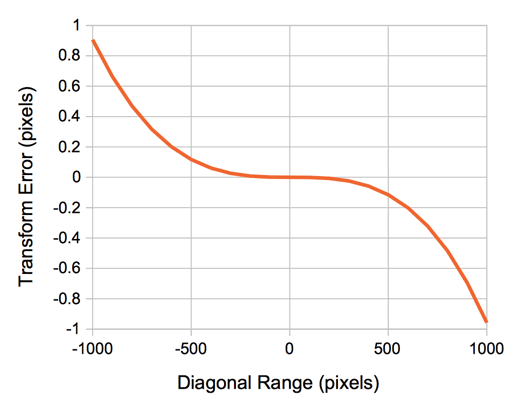Figure: X-Direction Error of the Transformation Functions versus Diagonal Distance from Pattern Origin.

The error in y is identical when plotted versus x-coordinate. The image extends only to ±350 pixels. The error at the corners of our image area is 0.4 pixels. Even in this extreme example, the average error will be less than 0.1 pixels, which is an error we are willing to accept in return for the computational efficiency of our sequential transformations.

## Large Rotations

[16-NOV-17] If our rasnik images are rotated by more than 150 mrad with respect to the image sensor rows and columns, the analysis is likely to fail. If we want to analyze a rasnik image that is rotated by 30° or 45°, we rotate the image by −30° or −45° before analysis. We make sure that our analysis bounds enclose only rotated mask squares, and no blank regions introduced by the rotation.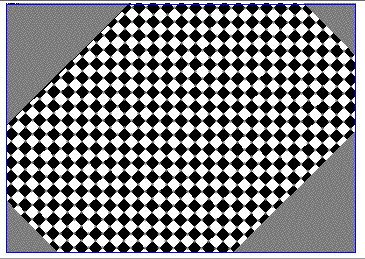Figure: Blank Regions Introduced by Rotation. The greater the difference between the width and height of the image, the more of the image we lose with rotation, and the larger the blank regions.

Starting with LWDAQ 8.4.1, the Rasnik Instrument provides the analysis_rotation_mrad parameter in its Info Panel. Use this parameter to specify the nominal rotation of the rasnik pattern in the counter-clockwise direction. The analysis rotates the image clockwise by this angle about the center of the analysis bounds, transforms the reference point into the rotated image coordinates, performs analysis, displays the rotated analysis on the original image, and adds the nominal rotation to the measured rotation to obtain its final result.

We set up a 360-° rotation stand with precision ±0.5 mrad. We mount upon the stand a 30-mm square rasnik mask. We clamp to our table an ICX424AL camera to view the rasnim mask so that the mask image is close to 0° when the stage reads 0°. We rotate the mask by ±50°. At each rotation we set analysis_rotation_mrad to the angle on our stage and capture an image.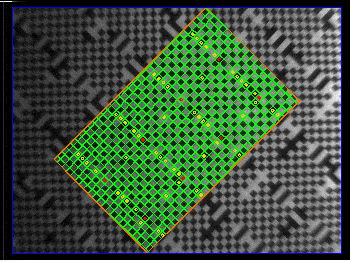Figure: Mask With Large Rotation, Analysis Overlayed.

We plot the difference between the rasnik-measured rotation and stage rotation.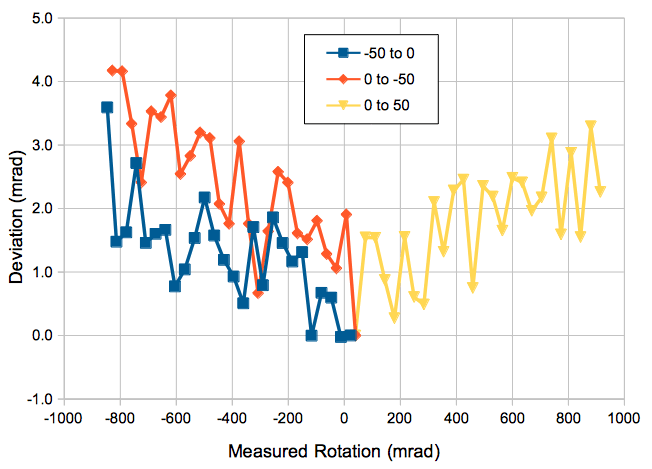Figure: Accuracy of Large Rotation Measurement.

We choose an ICX424AL image with 45° rotation and vary the pre-rotation constant we use to direct the analysis. We obtain the following plot of measured rotation versus pre-rotation.Figure: Effect of Pre-Rotation Upon Measured Rotation. We use an ICX424 image with a rasnik image rotated by 45° = 785 mrad.

So far as we can tell, our error in measuring large rotations is dominated by the error we introduce when we rotate the image in software.

[22-AUG-16] The rotation of the rasnik image we perform with the rotate operation of the lwdaq_image_manipulate routine. To test the accuracy of the rotation algorithm, we use the following code to create a simulated rasnik image with no rotation, rotate the image with the algorithm from −150 mrad to 150 mrad, and apply rasnik analysis.

```lwdaq_image_create -width 400 -height 400 -name sim
lwdaq_image_manipulate sim rasnik "200.0 200.0 20.0 20.0 0 10.0 1.0"
catch {image delete p}
image create photo p
destroy \$f.i
label \$f.i -image p
pack \$f.i
for {set rot -150.0} {\$rot <= +150.0} {set rot [expr \$rot + 10.0]} {
lwdaq_image_manipulate sim rotate 200 200 [expr \$rot/1000.0] -name newimage
lwdaq_image_manipulate newimage none -left 30 -right 370 -top 30 -bottom 370
set result [lwdaq_rasnik newimage -pattern_only 1]
LWDAQ_print \$t "\$rot \$result"
lwdaq_draw newimage p -intensify exact
LWDAQ_update
if {![winfo exists \$t]} {break}
}```

We obtain the following errors in pattern position. We are subtracting the known, fixed origin position and square sizes from the rasnik analysis measurements of these same parameters. Any offset in the measurement will appear in the graph.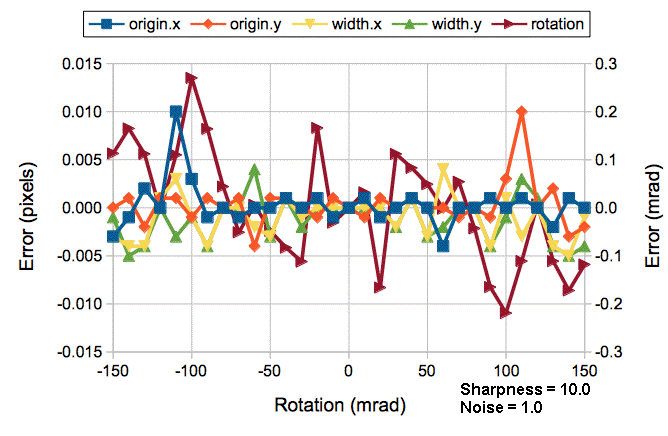Figure: Pattern Measurement Error versus Rotation for Simulated Image Rotated by Rotation Algorithm.

If our pixels are 10μm square, the rotation algorithm introduces less than 0.1 μm error. Errors with a dim, blurred image with significant noise are roughly ten times larger.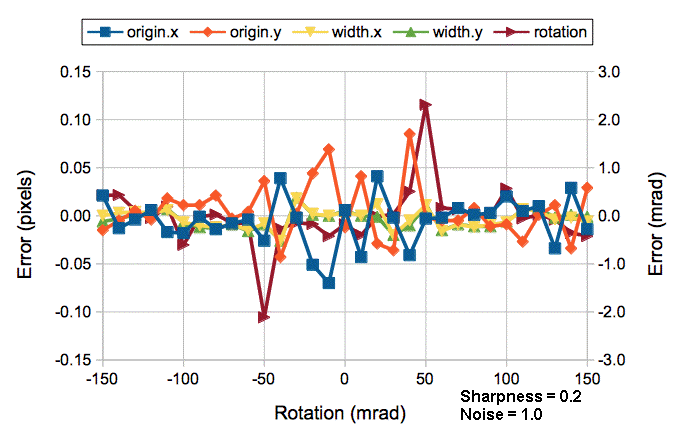Figure: Pattern Measurement Error versus Rotation for Poor Image Rotated by Rotation Algorithm. The simulated image has sinusoidal intensity variation with noise 8% of the sinusoidal amplitude.

[08-AUG-08] We generate rasnik patterns with rotation π/4±0.15 rad in 10-mrad steps. Each image we rotate by −&pi/4 before we apply rasnik analysis. We obtain the rasnik measurement, then rotate by −π/4 to display the results on the screen. Cut and paste the following script into the Toolmaker to reproduce our results.

```set wd 400
set ct [expr \$wd/2.0]
set nr [expr 3.1415926536/4.0]
set cl 30
lwdaq_image_create -width \$wd -height \$wd -name sim
catch {image delete p}
image create photo p
destroy \$f.i
label \$f.i -image p
pack \$f.i
for {set rot -30.0} {\$rot <= +30.0} {set rot [expr \$rot + 2.0]} {
lwdaq_image_manipulate sim rasnik "\$ct \$ct 20.0 20.0 [expr \$nr*1000 + \$rot] 10.0 1.0"
lwdaq_image_manipulate sim rotate -\$nr \$ct \$ct \
-name newimage -left \$cl -top \$cl -right [expr \$wd - \$cl] -bottom [expr \$wd - \$cl]
set result [lwdaq_rasnik newimage -pattern_only 1]
lwdaq_image_manipulate newimage rotate \$nr \$ct \$ct -name newimage
lwdaq_image_manipulate sim transfer_overlay newimage
lwdaq_draw sim p -intensify exact
LWDAQ_print \$t "\$rot \$result"
LWDAQ_update
if {![winfo exists \$t]} {break}
}```

At rotation π/4−0.06 rad, we see large errors due to confusion over which edge is which. We restrict ourselves to ±30 mrad and obtain the following errors with rotation.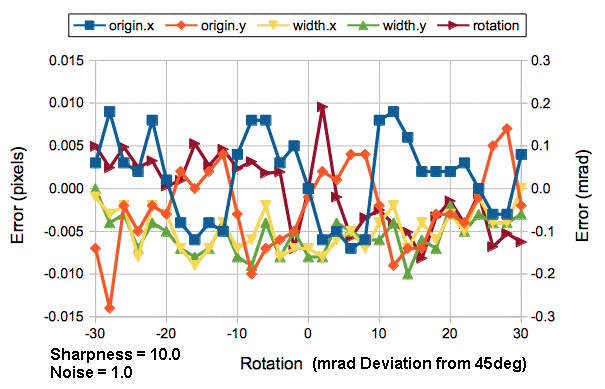Figure: Pattern Measurement Error versus Rotation for 45° Nominal Rotation. For a screen shot of one of the images, see here.

For 10-μm pixels, we will obtain the rasnik measurement to 0.1 μm accuracy even with a nominal rotation of 45°.

[10-AUG-16] When rotating an image, we must make sure the analysis region does not include any of the blank regions introduced by the rotation. We need an automatic way to determine how much to move the analysis boundaries for a given rotation. In the following derivation, we assume that we want the height and width of the boundaries to be reduced by the same amount.Figure: Boundary Reduction Required by Rotation.

The above formula assumes that the width of the image sensor is greater than or equal to the height, and that the rotation angle is greater than zero. If the height is greater than the width, we reverse the role of width and height in the formula. If the angle is negative, we take its absolute value. The graph below shows how far we must move the analysis borders for various image sensor dimensions and rotations.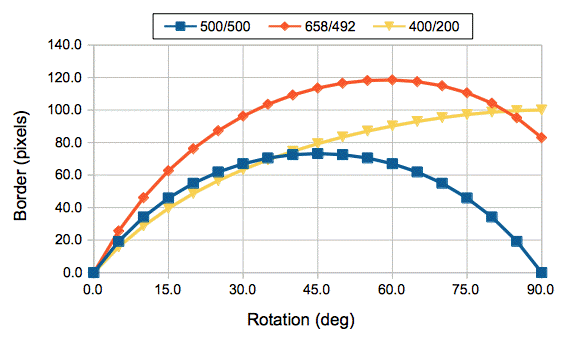Figure: Border Displacement versus Rotation. Various values of width/height. Units are pixels. The 658/492 matches the ICX424 image with our standard analysis boundaries.

Our ICX424 image, when rotated by 45° requires that we bring in all the borders by 114 pixels, which reduces the width of the analysis bounds from 658 to 430 and the height from 492 to 264.

We apply analysis to image Rasnik_blurred.gif image, which we keep in the image library we bundle with LWDAQ. We vary analysis_rotation_mrad from −150 to 150 mrad in 1-mrad steps. At 150 mrad, we get the following display of results.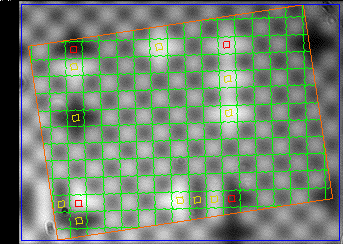Figure: Analysis Result Display, For Nominal Rotation 150 mrad. Square size is 170 μm, pixel size 10 μm. The image was taken from a 16-m rasnik insturment.

The orange rectangle is the analysis bounds used in the rotated image where analysis takes place. For each nominal rotation, we are rotating the image prior to analysis. With the image above, the standard devition of x is 1.0 μm and of y is 1.6 μm. The standard deviation of rotation is 2 mrad. We apply rasnik analysis with nominal rotation 0 mrad and 50 mrad to 552 images from the ATLAS End-Cap Alignment System. The standard deviation of the difference in x and y is 1.0 μm and in rotation is 0.5 mrad. (Also, when we cmompare LWDAQ 7.9.6 and LWDAQ 8.4.1 with 0 mrad, the results are identical.)

## Subroutines

At the bottom of rasnik.pas is a routine called rasnik_analyze_image.

```function rasnik_analyze_image(ip:image_ptr_type;
orientation_code:integer;
reference_x_um,reference_y_um,square_size_um,pixel_size_um:real):
rasnik_ptr_type;```

The routine takes six parameters. The first, ip, is a pointer to an image_type structure. We describe the image_type and all other structures defined by our code in the Data Types section below. The image_type contains a two-dimensional array of eight-bit gray-scale pixels, as well as auxilliary information about the image.

The orientation_code parameter tells rasnik_analyze_image the true orientation of the mask. Here are the values of orientation_code defined in rasnik.pas.

```rasnik_mask_orientation_nominal=1;
rasnik_try_all_orientations=0;```

The square_size parameter gives the size of the squares in the rasnik mask. We assume they are square, not rectangular. Likewise, the pixel_size parameter gives the size of the pixels in the image sensor.

The rasnik_analyze_image procedure returns a rasnik_ptr_type, which is a pointer to a newly-created rasnik_type structure. The rasnik_type contains the results of rasnik analysis, as well as the input parameters, but not the image_type itself. There is enough information in the rasnik_type for us to re-calculate the rasnik measurement with respect to a different reference point, using a routine like rasnik_shift_reference_point. We describe the fields of the rasnik_type in the next section.

The body of rasnik_analyze_image is a sequence of subroutines.

```iip:=image_grad_i(ip);

These two routines create two more images giving us the absolute value of the intensity derivative in the horizontal (i) and vertical (j) directions, as we describe above. Both routines operate upon an image_type and return a new image_type. You will find the routines defined in image_manip.pas. The derivative images show the horizontal and vertical edges in the image.

`pp:=rasnik_find_pattern(iip,jip,false);`

This step finds the approximate pattern using slices of the derivative images, one-dimensional fourier analysis (see above), and smoothing of the intensity profile (see above). The routine takes as input the two derivative images and a show_fitting flag. When true, this flag instructs the routine to show stages of analysis via the graphical user interface. The routine returns a pointer to a new rasnik_pattern_type structure, which defines the phase, period, and rotation of a chessboard pattern overlayed upon the image. We describe the rasnik_pattern_type in the next section.

`rasnik_refine_pattern(pp,iip,jip,false);`

This step refines the approximate pattern by fitting lines to edge pixels (see above). The routine operates upon an existing rasnik_pattern_type pointed to by pp.

`rasnik_adjust_pattern_parity(ip,pp);`

So far, the analysis has looked only at edges in the image. It has determined the orientation and magnification of the rasnik pattern, and determined a viable offset for this pattern that will place its edges in the correct place on the two derivative images. But the analysis has not yet made sure that the white squares of its pattern coincide with the white squares in the image. The rasnik_adjust_pattern_parity routine looks at the original image, as pointed to by ip, and adjusts the pattern, as pointed to by pp, so that the white squares in the pattern match the white squares in the image.

`rasnik_identify_pattern_squares(ip,pp);`

This step is the first to use of the squares field of the rasnik_pattern_type pointed to by pp. The squares field is a two-dimensional array with an element for each square of the pattern. The routine marks the squares in the squares array that correspond to squares in the image that lie within the image's analysis boundaries.

`rasnik_identify_code_squares(ip,pp);`

This steps marks the squares in the squares array that are out of parity in the image. The out of parity squaresa are the rasnik code squares. The routine marks as pivot squares any code squares that are at the intersection of lines and columns of code squares.

`rasnik_analyze_code(pp,orientation_code);`

This step analyses the squares array the rasnik_pattern_type pointed to by pp. It records records the line and column codes of each valid pivot squares. The orientation_code parameter tells the analysis whether it should guess the orientation or use a particular orientation.

`rp:=rasnik_from_pattern(ip,pp,reference_x,reference_y,square_size_um,pixel_size_um);`

The rasnik_from_pattern routine takes the pattern pointed to by pp, which has all its pivot squares marked with their line and column codes, and calculates the final rasnik measurement. It stores the measurement in a new rasnik_type structure and returns a pointer to that structure. The referencde_x and reference_y parameters are used only when the reference_code is equal to the value rasnik_reference_image_point. We need to pass ip to this routine because we need to know the dimensions of the image and its analysis bounds for various values of reference_code.

```dispose_rasnik_pattern(pp);
dispose_image(iip);
dispose_image(jip);```

These steps free up the memory used by the pattern and the derivative images.

`rasnik_analyze_image:=rp;`

The final step is to return a pointer to the rasnik_type that contains the rasnik measurement. This rasnik_type structure was allocated dynamically, so the calling procedure must call dispose_rasnik to de-allocate the structure.

## Data Types

Here we describe the Pascal data types used by our rasnik analysis routines. If your plan is to call our rasnik routines from C, be assured that the Calling from C section tells you how to translate between standard C data types and our Pascal data types.

The rasnik_analyze_image function uses three data types for its input parameters: image_ptr_type, integer, and real. It returns a pointer to a newly-allocated rasnik_type. Inside rasnik_analyze_image, we see a rasnik_pattern_type used by several stages of the analysis. We describe all these types below.

### Simple Types

Some of our Pascal types are simple enough that they have equivalent types in other languages, like C and Fortran. We describe these simple types here.

An integer is a four-byte signed integer. A real is an eight-byte floating-point number.

An xy_point_type is a pair of real-valued coordinates, defined in utils.pas. We also have ij_point_type, which is a pair of integer-valued coordinates.

```xy_point_type=record x,y:real; end; {size is 16 bytes}
ij_point_type=record i,j:integer; end; {size is 8 bytes}```

A byte is an unsigned eight-bit integer, value 0 to 255. A boolean is a binary variable. A solitary boolean takes up one byte, but a boolean field in a larger data structure can take up four bytes. Packing more than one boolean into a single byte will work on some compilers. On MacOS, we can pack sixteen booleans into two bytes, but we cannot pack eight booleans into one byte.

To represent rectangles in an image, we use our ij_rectangle_type.

`ij_rectangle_type=record top,left,bottom,right:integer; end;`

The four fields of the rectangle are the edges of a rectangle. The size of the structure is 16 bytes.

### Strings

Note: The following applies to LWDAQ 9 or earlier. We have yet to prepare and write up the string types of our LWDAQ 10 routines.

The short_string and long_string types are defined in utils.pas, with lines like those below.

```const
short_string_length=2000;
long_string_length=300000;
type
short_string = string(short_string_length);
long_string = string(long_string_length);```

A Pascal string is similar to a C string. Instead of being null-terminated, it has a length parameter. The Pascal string is an example of a Pascal schema type, whose dimensions are determined by one or more parameters. A more complicated schema type is the image_type.

To translate between Pascal strings and C or Fortran strings, you need dedicated routines. We will describe such routines below.

### image_type

Note: The following applies to LWDAQ 9 or earlier. We have yet to prepare and write up the image types of our LWDAQ 10 routines.

The first input to rasnik_analyze_image is an image_ptr_type, which is a pointer to an image_type. The image_type is defined in image.pas. It is a Pascal schema type. A schema type's dimensions depend upon its discriminants. An image_type's discriminants are the number of lines, j_size, and the number of columns, i_size you see in the image_type definition below.

```image_type(j_size,i_size:integer)=record
intensity:array [0..j_size-1,0..i_size-1] of byte;
overlay:array [0..j_size-1,0..i_size-1] of byte;
analysis_bounds:ij_rectangle_type;
average,amplitude,maximum,minimum:real;
name:short_string;
intensification:integer;
results:short_string;
end;
image_ptr_type=^image_type;```

We specify j_size and i_size when we creat a new image_type. We create an image_type with the following Pascal instruction.

`ip:=new(image_ptr_type,j_size,i_size);`

In this Pascal instruction, the ip variable is a pointer to an image_type, which we call an image_ptr_type. The number of rows in the image is j_size, and the number of columns is i_size. In our code, we tend to use j and i to indicate row and column number respectively.

There are two pixel arrays in an image_type. The intensity array contains the pixels of the image. In the current version of our code, these pixels are each one-byte gray-scale intensities. The overlay array has the same dimensions as the intensity array. Each overlay pixel is also one byte.

The overlay contains a picture, graph, or set of marker lines that we draw on top of the intensity when we display the image for the user. When overlay[j,i] is not equal to clear_color, we change the color of pixel intensity[j,i] in the image display. The color with which we draw overlay[j,i] depends upon its value. You will find a list of colors and their corresponding values in image.pas. The ranik routines draw into the overlays of the original image and the derivative images, and so make it possible for our LWDAQ Software to display the results and stages of anlysis in such a way that we can spot problems and make corrections.

The analysis_bounds field is an ij_rectangle_type that defines a rectangle in the image within which image analysis is to take place. Pixels outside the analysis_bounds will be ignored. The ij_rectangle_type is defined in utils.pas as follows:

`ij_rectangle_type=record top,left,bottom,right:integer; end;`

The average, amplitude, maximum, and minimum fields are real numbers. We use these variables to store properties of the image during certain image analysis procedures. But we do not use them in rasnik analysis.

The name field holds a name for the image, which we use to organise and sort the LWDAQ Software's internal image list. The intensification field selects one of sevaral types of intensification available for screen display of the gray-scale image.

```no_intensify=0;
mild_intensify=1;
strong_intensify=2;
exact_intensify=3;```

The results field is a string we use to pass data acquisition parameters to certain image analysis routines. The only use our rasnik analysis makes of any of these three fields is to use the name field when setting up its diagnostic displays.

### rasnik_type

Note: The following applies to LWDAQ 9 or earlier. We have yet to prepare and write up the rasnik types of our LWDAQ 10 routines.

The result returned by rasnik_analyze_image is a pointer to a rasnik_type, defined in rasnik.pas as follows.

```rasnik_type=record
valid:boolean;{no problems encountered during analysis}
magnification_x,magnification_y:real;{magnification along pattern coords}
skew_x,skew_y:real;{skew in x and y directions in rad/m}
reference_point_um:xy_point_type;{point in image, um from top-left corner}
pixel_size_um:real;{width of a sensor pixel in um}
end;
```

We have already defined each of the simple types used in the rasnik_type structure. Because the rasnik_type consists only of fixed-length fields, it is possible to pass a rasnik_type directly from Pascal to C or Fortran. But we must make sure our compilers agree upon how to arrange the structure fields in memory. The offset of each field from the base address of the rasnik_type is given by the following table.

FieldTypeOffset
validboolean0
magnification_xreal24
magnification_yreal32
skew_xreal40
skew_yreal48
slantreal56
rotationreal64
errorreal72
reference-pointxy_point_type84
square_size_umreal100
pixel_size_umreal108
Table Address Offsets of rasnik_type fields.

The padding field ensures that all four-byte variables are on four-byte boundaries, and all eight-byte variables are on eight-byte boundaries. We do this because the newer versions of GPC will place the eight-byte variables on eight-byte boundaries whether we like it or not. The only way for our rasnik_type to be compatible with all versions of GPC and GCC is to force the eight-byte variables onto eight-byte boundaries regardless of the compiler.

### rasnik_pattern_type

Note: The following applies to LWDAQ 9 or earlier. We have yet to prepare and write up the rasnik types of our LWDAQ 10 routines.

The intermediate stages of rasnik analysis, as described above use a rasnik_pattern_type structure to store information about the image and the rasnik pattern.

```rasnik_pattern_type=record {based upon pattern_type of image_types}
valid:boolean;{most recent analysis yielded valid output}
padding:array [1..7] of byte;{force origin to eight-byte boundary}
origin:xy_point_type;{pattern coordinate origin in image coordinates}
pattern_x_width,pattern_y_width:real;{square width in pixels along pattern coords}
image_x_skew,image_y_skew:real;{derivative of line slope in rad/pixel}
image_x_width,image_y_width:real;{square separation in pixels along image coords}
error:real;{pixels rms in image}
extent:integer;{extent of pattern from center of analysis bounds}
x_code_direction,y_code_direction:integer;{directions of code increment}
analysis_center_cp:ij_point_type;{ccd coords of analysis bounds center}
analysis_width:real;{diagnonal width of anlysis bounds}
squares:rasnik_square_array_ptr_type;{array of rasnik squares}
more_padding:array [1..4] of byte;{force end to eight-byte boundary}
end;```

As with rasnik_type, the padding field makes sure that all the eight-byte variables are on eight-byte boundaries. Here are the address offsets of each field.

FieldTypeOffset
validboolean0
originxy_point_type8
rotationreal24
pattern_x_widthreal32
pattern_y_widthreal40
pattern_x_skewreal48
pattern_y_skewreal56
image_x_widthreal64
image_y_widthreal72
image_slantreal80
errorreal88
extentinteger92
x_code_directioninteger100
y_code_directioninteger104
analysis_center_cpij_point_type108
analysis_widthreal116
squaresrasnik_square_array_ptr_type124
Table: Address Offsets of rasnik_pattern_type fields. Total record size is 120 bytes.

The squares field is a pointer to a two-dimensional array of rasnik_square_type structures. This array contains an element for every square in the rasnik image, and allows every square to be classified as black or white, as a code square, as as a pivot square. We describe the the rasnik_square_type below, and the rasnik_square_array_type also.

The rasnik_find_pattern routine creates a new pattern_type with our new_rasnik_pattern routine. It fills in the valid, origin, rotation, pattern_x_width, pattern_y_width, image_x_width, image_y_width, and extent fields for use in subsequent routines like rasnik_refine_pattern and rasnik_adjust_pattern_parity.

### rasnik_square_type

Note: The following applies to LWDAQ 9 or earlier. We have yet to prepare and write up the rasnik types of our LWDAQ 10 routines.

A rasnik_square_type describes a single square in a rasnik pattern.

```rasnik_square_type=record
center_pp:xy_point_type; {center of square in pattern coordinates}
center_intensity:real; {average intensity of central region}
center_whiteness:real; {whiteness score, >0 for white, <=0 for black}
pivot_correlation:integer; {number of agreeing pivot squares}
x_code,y_code:integer; {the column and line codes for the pivot square}
display_outline:ij_rectangle_type; {a rectange for square marking}
is_a_valid_square:boolean; {within the analysis boundaries}
is_a_code_square:boolean; {out of parity with the chessboard}
is_a_pivot_square:boolean; {at intersection of code line and column}
end;
rasnik_square_ptr_type=^rasnik_square_type;```

The rasnik_square_type uses an array of bytes to pad its size to an eight-byte boundary. In fact, we leave a little extra padding to accomodate additional fields in the future.

FieldTypeOffset
center_ppxy_point_type0
center_intensityreal16
center_whitenessreal24
pivot_correlationinteger32
x_codeinteger36
y_codeinteger40
display_outlineij_rectangle_type44
is_a_valid_squareboolean60
is_a_code_squareboolean61
is_a_pivot_squareboolean62
Table: Address Offsets of rasnik_square_type fields. Total record size is 80 bytes.

We use the rasnik_square_type to form the elements of the array pointed to by the squares pointer in a rasnik_pattern_type.

### rasnik_square_array_type

Note: The following applies to LWDAQ 9 or earlier. We have yet to prepare and write up the rasnik types of our LWDAQ 10 routines.

A rasnik_square_array is a dynamically-allocated two-dimensional array of rasnik_square_type elements. The array provides an element for each square in a rasnik image. When we create the array, we specify its dimensions with the extent discriminant.

```rasnik_square_array_type(extent:integer)=
array [-extent..extent,-extent..extent] of rasnik_square_type;
rasnik_square_array_ptr_type=^rasnik_square_array_type;```

The square array is a Pascal schema type. We cannot duplicate a Pascal schema type in C in any reliable way, because the schema types contain header information of length that varies form one version of the Pascal compiler to the next. We can, however, duplicate rasnik_square_type in C, and we can specify squares in an array with their array indices. Our rasnik_square_get routine copies the contents of an element in a square array to a rasnik_square_type of your own. Our rasnik_square_put routine copies the contents of your own rasnik_square_type to an element in a square array. We describe these routines below.

When we first create a rasnik_pattern_type, the squares pointer is set to nil. The routine rasnik_identify_pattern_squares creates a two-dimensional array of rasnik_square_type just large enough to cover the rasnik squares in the image. A typical square array will have extent 19 and contain 1600 squares, each of size 80 bytes. The total array is 128 kBytes. But some rasnik images contain tiny squares, and our extent can be up to 39, in which case our array size is 512 kBytes.

## Calling from C

Note: The following applies to LWDAQ 9 or earlier. We have yet to prepare and write up the C interface for our LWDAQ 10 routines.

If you need to call our Pascal routines from C, we recommend you start by reading our Mixing Manual, which describes how to combine C and Pascal code into a single application.

### Examples

Note: The following applies to LWDAQ 9 or earlier. We have yet to prepare and write up the C interface of our LWDAQ 10 routines.

We will use example code to show you how to call our rasnik routines. So you must download and compile our example code to follow our discussion. Start by downloading and installing the latest version of our LWDAQ Software. You will find installation instructions for Linux, Windows, MacOS, and UNIX in our LWDAQ Manual. Install GPC (the GNU Pascal Compiler) on your system using our instructions. With any luck, our instructions will lead to a binary distribution of the compiler that you can unpack and install. If you are unlucky, you will have to compile GPC from its source code.

Once you have GPC and LWDAQ installed, go to the LWDAQ/Build directory and build our analysis library with the following command.

`make analysis`

You should now see a file called analysis.a in the Build directory. This static library contains all our analysis routines, including our rasnik routines, as well as the Pascal run-time library routines. Copy our C example programs, c.c, or our C++ example program, cpp.cpp. Put these in the LWDAQ/Build directory. You will have to over-write whatever c.c or cpp.cpp file is already present in the Build directory. Open a terminal window and go to the Build directory. On Windows, you will be opening a Cygwin terminal window, not a DOS terminal window. Enter the following commands.

```make c
./c.exe```

The make utility compiles c.c and links it to analysis.a to create a console program called c.exe. When you run the program, it asks you for a file name. Enter 0 for the default file in the Code directory. The program prints its results to the console. You can compile and run cpp.cpp the same way.

```make cpp
./cpp.exe```

The source files c.c and cpp.cpp illustrate how to define a rasnik_type and call routines from analysis.a.

### Initialization

Note: The following applies to LWDAQ 9 or earlier. We have yet to prepare and write up the C interface of our LWDAQ 10 routines.

Before you can use the routines in analysis.a, you must call analysis_init, the routine that initializes the Pascal run-time library and the variables in all the units embedded in analysis.a. In c.c and cpp.cpp you will see calls to the initialization routine, which you refer to in C as Analysis_Init

The hello_world routine, referred to in C as Hello_World prints a line to the console. You can use this routine to make sure that you have connected to analysis.a.

### Complete Analysis

Note: The following applies to LWDAQ 9 or earlier. We have yet to prepare and write up the C interface of our LWDAQ 10 routines.

We provide two routines that perform complete rasnik analysis. Both require that you define the rasnik_type in your source code. Here is the definition of rasnik_type from c.c.

```struct rasnik_type {
unsigned char valid;
double magx;
double magy;
double rot;
double error;
struct xy_point_type reference_point;
double square_size;
double pixel_size;
};```

Our rasnik_analyze_file routine accepts a file name in the form of a pointer to a null-terminated C-string. The file must be an image in our DAQ image format. The routine returns a pointer to a newly-allocated rasnik_type. The fields of the rasnik_type contain the result of the rasnik analysis. The line from c.c that calls the rasnik analysis is:

```
rp=Rasnik_Analyze_File(file_name,0,2,0,0,120,10);
```

The rasnik_analyze_file routine is declared in rasnik.pas. Its numerical parameters are identical to those of rasnik_analyze_image, which we described above. The only difference between the two routines is that one takes a pointer to an image and the other takes a pointer to a C string.

If you have your image in memory as an array of gray-scale single-byte pixels, you can analyze the image with rasnik_analyze_image, but first you have to create an image_type with your array as its contents. In images.pas, you will find image_from_contents, which takes a pointer to your intensity array and several other parameters to specify the dimensions of the image and its analysis boundaries.

```function image_from_contents(intensity_ptr:pointer;
width,height,left,top,right,bottom:integer;
results,name:CString):image_ptr_type;```

The routine copies your intensity array and returns a pointer to an image_type. Your C-code can use a generic pointer variable to hold the pointer returned by the routine, and so pass the same pointer to subsequent routines from our analysis library. There should be no need for your C-code to refer to elements in the image_type. Now you can analyze the image with rasnik_analyze_image. The cp variable is a pointer to your intensity array. The values we pass to image_from_contents in thie example are correct for image Sixteen_Meter.daq.

```char *ip
ip=Image_From_Contents(cp,344,244,20,2,343,243,"","Sixteen_Meter");
struct rasnik_type *rp;
rp=Rasnik_Analyze_Image(ip,0,2,0,0,120,10);```

If you want to read a DAQ image off disk and into an image_type, you use read_daq_image, which is defined in images.pas.

```char *ip

When you are finished with rp^ (the rasnik_type pointed to by rp), you dispose of it with the following line.

`Dispose_Rasnik(rp);`

You may wish to store your image in our DAQ image format. You can do that with write_daq_file, which is declared in images.pas.

`Write_Image_File(file_name,ip);`

When you are done with ip^, dispose of it with dispose_image.

`Dispose_Image(ip);`

You will find other routines in images.pas that we intend for use with C programs. The table below lists the routines and their purpose.

RoutinePurpose
New_Imagecreates a new image_type
Dispose_Imagedisposes of an image_type
Image_From_Daqconverts DAQ block into an image_type
Daq_From_Imageconverts and iamge_type into a DAQ block
Write_Daq_Filewrites an image_type to a DAQ file
Image_From_Contentsconverts a pixel array into an image_type
Contents_From_Imageextracts the pixel array from an image_type
Table: Routines Exported by images.pas for C Programs. For explanations of each routine, consult the source code.

The names given in the table are the export names. The Pascal names are the same, but without capital letters. In C, you must use the capitalized names with the external command. A DAQ block is a block of data representing an image in our DAQ image format. When you store a DAQ block to disk, you create a DAQ image file.

### Partial Analysis

Note: The following applies to LWDAQ 9 or earlier. We have yet to prepare and write up the C interface of our LWDAQ 10 routines.

Suppose you have your own routine for finding the chessboard pattern in an image, but you do not have routines that interprets the code squares. You can use a rasnik_pattern_type structure to pass your chessboard pattern to our code-analyzing routines, and so obtain a rasnik measurement. Alternatively, you might be writing your own code-analyzing routines, and you would like to use our routines to find the chessboard pattern. You can use a rasnik_pattern_type structure to receive the chessboard pattern from our pattern-finding routines.

To help you perform these or any other partial analysis using our libraries, we will show you how to call every stage of rasnik analysis from C, and examine the intermediate results in C also. First, we list the rasnik routines available in analysis.a. These are all defined in rasnik.pas.

RoutinePurpose
New_Rasnikcreate new rasnik_type
Dispose_Rasnikdispose of rasnik_type
New_Rasnik_Patterncreate new rasnik_pattern_type with empty square array
Dispose_Rasnik_Patterndisposes of rasnik_pattern_type
Rasnik_Analyze_Imagecomplete analysis of image_type
Rasnik_Analyze_Filecomplete analysis of DAQ file
Rasnik_Find_Patternfind rasnik pattern in image
Rasnik_Refine_Patternrefine a rasnik pattern
Rasnik_Adjust_Pattern_Paritycorrect the parity of a rasnik pattern
Rasnik_Identify_Pattern_Squaresidentify valid squares rasnik pattern
Rasnik_Identify_Code_Squaresidentify code squares in rasnik pattern
Rasnik_Analyze_Codeanalyze code squares in rasnik pattern
Rasnik_From_Patternobtain rasnik measurement from rasnik pattern
Rasnik_Get_Squarecopy a rasnik_square_type out of a square array
Rasnik_Put_Squarecopy a rasnik_square_type into a square array
Table: Routines Exported by rasnik.pas for C Programs. Routines that work on patterns use the rasnik_pattern_type. Comments in the source code explain each routine, but we provide additional explanation below.

The first rasnik routine we call is rasnik_find_pattern. This routine uses two intensity-gradient images, or derivative images. One image is the absolute value of the intensity gradient in the horizontal, or i, direction. Positive i is left to right. The other image is the absolute value of the intensity gradient in the vertical, or j direction. Positive j is top to bottom. The routines you need to obtain these gradients are in image_manip.pas

RoutinePurpose
Table: Routines Exported by image_manip.pas for C Programs.

The C-code at the end of c.c reads the image off disk and analyzes it in stages. We display the prominant fields of the pattern during its refinement, and extract a square from the pattern's square array in and display its fields also.

### Coordinate Systems

Our code uses three coordinate systems. We call them ccd, image, and pattern coordinates. Our transforms.pas unit defines the three coordinate systems and declares routines to transform between them.

Our ccd coordinates specify a pixel in an image. They are named after the type of image sensor we used for most of our cameras. The letters CCD stand for Charge-Coupled Device. Our CCD images are rectangular with square pixels. The pixels are arranged in rows and columns. We specify a pixel in the image with its column and row number. A ccd point has the form (i,j) where i is the column number and j is the row number. Pixel (0,0) is the top-left pixel. Column number increases from left to right and row number increases from top to bottom. CCD Coordinates are therefore left-handed. We use ij_point_type for points in CCD Coordinates. You will find ij_point_type defined in our utils unit.

Our image coordinates specify a point in an image. An image point has the form (x,y), where x and y are real numbers, x is horizontal distance from left to right and y is vertical distance from top to bottom. The units of both x and y are pixels. Image point (0,0) is at the top-left corner of the top-left pixel. Point (1,1) is the bottom-right corner of the top-left pixel, and also the top-left corner of the second pixel in the second row.

We have constants ccd_origin_x and ccd_origin_y that define the location of the ccd coordinate origin in image coordinates. The values declared in our current version of rasnik.pas are 0.5 and 0.5, which places the ccd origin at the center of the top-left pixel.

Our pattern coordinates specify a point in a pattern superimposed on an image. Pattern points are ot the form (x,y), where x and y are the real. We assume the pattern is periodic in two orthogonal directions. A chessboard rasnik mask is such a pattern, and so is a sequence of encoder lines. The chessboard has a finite period in two directions, while the encoder lines have a finite period in one direction and a large period in the other direction. The x-axis is parallel to one direction in the orthogonal pattern, and the y-axis is parallel to the other direction. Pattern coordinates are left-handed for better cooperation with ccd and image coordinates, both of which are left-handed.

We define a pattern coordinate system with five numbers grouped in a pattern_type record. These numbers represent a translation, rotation, and scaling in two directions between image coordinates and pattern coordinates.

```pattern_type=record
valid:boolean;{valid pattern}
padding:array [1..7] of byte; {force origin field to eight-byte boundary}
origin:xy_point_type; {pattern coordinate origin in image coordinates}
pattern_x_width:real; {scaling factor going from pattern x to image}
pattern_y_width:real; {scaling factor going from pattern y to image}
image_x_skew:real; {derivative of horzontal edge slope with x in rad/pixel}
image_y_skew:real; {derivative of vertical edge slope with y in rad/pixel}
image_slant:real; {non-perpendicularity of pattern in image in rad}
end;```

The pattern_type structure matches that of the first few fields of the rasnik_pattern_type. The origin field is an xy_point_type that gives the image coordinates of the pattern coordinate origin. The origin will have some significance in the pattern. In the case of rasnik analysis, the origin lies at the top-left corner of a chessboard square. In our wire-finding code, the origin lies at the center of a wire shadow. We choose the origin square so that the pattern coordinate origin is near the center of the analysis bounds. This choice minimizes the errors that arise from rotation and skew in the image. The rotation field gives the rotation of the pattern coordinates in the anticlockwise direction with respect to image coordinates. The pattern_x_width field gives the length of one period of the pattern along the pattern x-axis, as measured in image coordinates. Imagine the pattern x-axis inclined at a slight angle with respect to the image rows, and therefore to the image x-axis. A square in the pattern has one edge parallel to the pattern x-axis. The length of this edge, as measured in the image coordinates, is pattern_x_width. We have pattern_y_width as well, because a pattern can, in general, be rectangular in its geometry.

We assume the mask itself contains perfect, uniform, squares. But the projection of the mask onto the image sensor can be both skewed and stretched. If the mask is rotated about its x-axis, for example, the squares will appear narrower in the x-direction than in the y-direction. Our pattern accounts for this difference with its two values of pattern width. If the distance from the mask to the lens is such that the rotation causes a significant change in the opject distance of the mask across the image, the width and height of the squares will change across the image. Our pattern accounts for this variation with its two skew parameters.

The pattern coordinate origin is the top-left corner of the origin square in a chessboard pattern. Point (1,0) marks the top-right corner of the same square, and also the top-left corner of the square to its right. Here were defining right and left as +ve and −ve in the x-direction, and top and bottom as +ve and −ve in the y-direction. Point (0,1) is the bottom-left corner of the same square, and the top-left corner of the square immediately below. Moving around through a chessboard pattern is easy in pattern coordinates, because we simply add one to our x-coordinate to move to the square on the right, and add one to our y-coordinate to move to the square below. Transforming between image and pattern coorsinates is more complicated, because of the asymmetry, rotation, skew, and slant between the image and the object. Here are the two routines we use to move between the two coordinate systems. For a derivation of the effecty of skew upon the transform, see Skewed Images.

```function p_from_i(point:xy_point_type;pattern_ptr:pointer):xy_point_type;
const min_slant_cosine=0.5;
var p:pattern_ptr_type;q,r:xy_point_type;slant,c,s,cc:real;
begin
p:=pattern_ptr_type(pattern_ptr);
with p^ do begin
q.x:=(point.x-origin.x);
q.y:=(point.y-origin.y);
r.x:=q.x/(1 + image_x_skew*q.x)/(1 + image_y_skew*q.y);
r.y:=q.y/(1 + image_x_skew*q.x)/(1 + image_y_skew*q.y);
if slant>max_slant then slant:=max_slant;
c:=cos(image_slant/2);
s:=sin(image_slant/2);
cc:=c*c-s*s;
q.x:= (c*r.x - s*r.y)/cc;
q.y:= (-s*r.x + c*r.y)/cc;
r.x:=q.x/pattern_x_width;
r.y:=q.y/pattern_y_width;
c:=cos(rotation);
s:=sin(rotation);
q.x:=r.x*c - r.y*s;
q.y:=r.x*s + r.y*c;
end;
p_from_i:=q;
end;

function i_from_p(point:xy_point_type;pattern_ptr:pointer):xy_point_type;
var p:pattern_ptr_type;q,r:xy_point_type;slant,c,s:real;
begin
p:=pattern_ptr_type(pattern_ptr);
with p^ do begin
c:=cos(rotation);
s:=sin(rotation);
q.x:=  point.x*c + point.y*s;
q.y:= -point.x*s + point.y*c;
r.x:=q.x*pattern_x_width;
r.y:=q.y*pattern_y_width;
if slant>max_slant then slant:=max_slant;
c:=cos(image_slant/2);
s:=sin(image_slant/2);
q.x:=  c*r.x + s*r.y;
q.y:=  s*r.x + c*r.y;
r.x:=q.x
/(1 - image_x_skew*q.x)
*(1 + image_y_skew*q.y/(1 - image_y_skew*q.y))
+ origin.x;
r.y:=q.y
/(1 - image_y_skew*q.y)
*(1 + image_x_skew*q.x/(1 - image_x_skew*q.x))
+ origin.y;
end;
i_from_p:=r;
end;```

The pattern_type is the basis of any record used with the pattern coordinate tranform routines defined in this unit. But the pattern_type is not public. All transform routines refer to patterns through generic pointers. When another unit declares its own pattern record for use with the routines declared in this unit, the new record must begin with the same fields as pattern_type, so these fields may be referred to through a pointer as if the new record were a genuine pattern_type. See rasnik_pattern_type in rasnik.pas for an example of such a pattern type.

The rasnik_pattern_type places its pattern coordinate origin at the top-left corner of a rasnik square. Any rasnik square will do, but our analysis tries to pick one that's near the center of the image. If p is a rasnik_pattern_type, the point (0,0) in pattern coordinates lies at point p.origin in image coordinates. Just to be clear, we'll say the same thing again: the origin field is an xy_point_type giving the image coordinates of the pattern coordinate origin. The rotation field gives the rotation of the pattern x-axis with respect to the image x-axis, with counter-clockwise rotation being positive. The pattern_x_width parameter is the distance between pattern points (0,0) and (1,0) after they have been transformed into image coordinates. When the pattern rotation is small, pattern_x_width gives the length of the near-horizontal sides of the squares. The pattern_y_width parameter is the length of the near-vertical sides of the squares.

### Custom Analysis

You can write your own routine to find the rasnik pattern in an image, and make use of our code square analysis afterwards. To implement your own pattern-finding routine, give it the same structure and output as our rasnik_find_pattern routine. Create a new pattern_type and fills the following fields: valid, origin, rotation, pattern_x_width, pattern_y_width, image_x_width, image_y_width, and extent. You may now pass this pattern_type to our rasnik_refine_pattern, where it will be refined, or you can skip our refining routine and go straight to rasnik_adjust_pattern_parity and proceed all the way through code square analysis to rasnik_from_pattern.